# NCERT Exemplar Class 10 Science Solutions for Chapter 10 - Light Reflection And Refraction

## NCERT Exemplar Solutions Class 10 Science Chapter 10 – Free PDF Download

NCERT Exemplar Solutions for Class 10 Science Chapter 10 Reflection and Refraction will boost your confidence by providing you with the different questions that can be asked on the topic Reflection and Refraction. In chapter 10, students will understand the phenomena of reflection and refraction of light and at the same time, they will learn about the basic concepts related to some of the optical phenomena occurring in nature. Students will also understand the reflection of light by spherical mirrors and refraction of light and other concepts like the mirror formula, refractive index, lens formula and more.

While there are many topics to cover, we are providing free NCERT Exemplar Solutions for Class 10 Science Chapter 10 here. These exemplars will facilitate easy learning and students will be able to get clarity on all the concepts and topics given in this chapter. Besides, these Exemplar Solutions that are given here act as a very useful reference tool with which students will further get to know all the important chapter topics, practice questions, complete revisions and ultimately be well prepared to write their exams.

Students can take a quick look of Class 10 Science Chapter 10 NCERT Exemplar below.

### Download PDF of NCERT exemplar for Class 10 Science Chapter 10 – Light Reflection and Refraction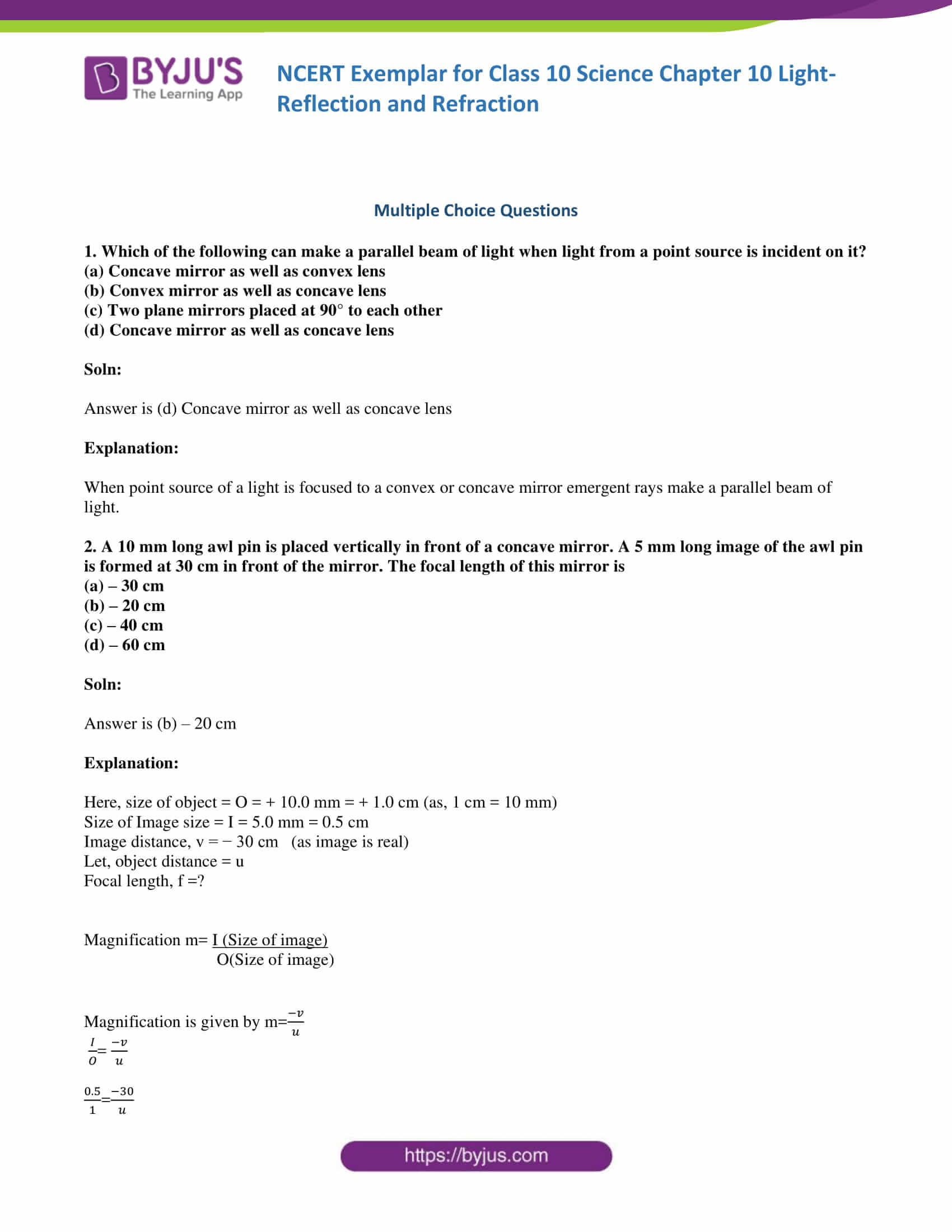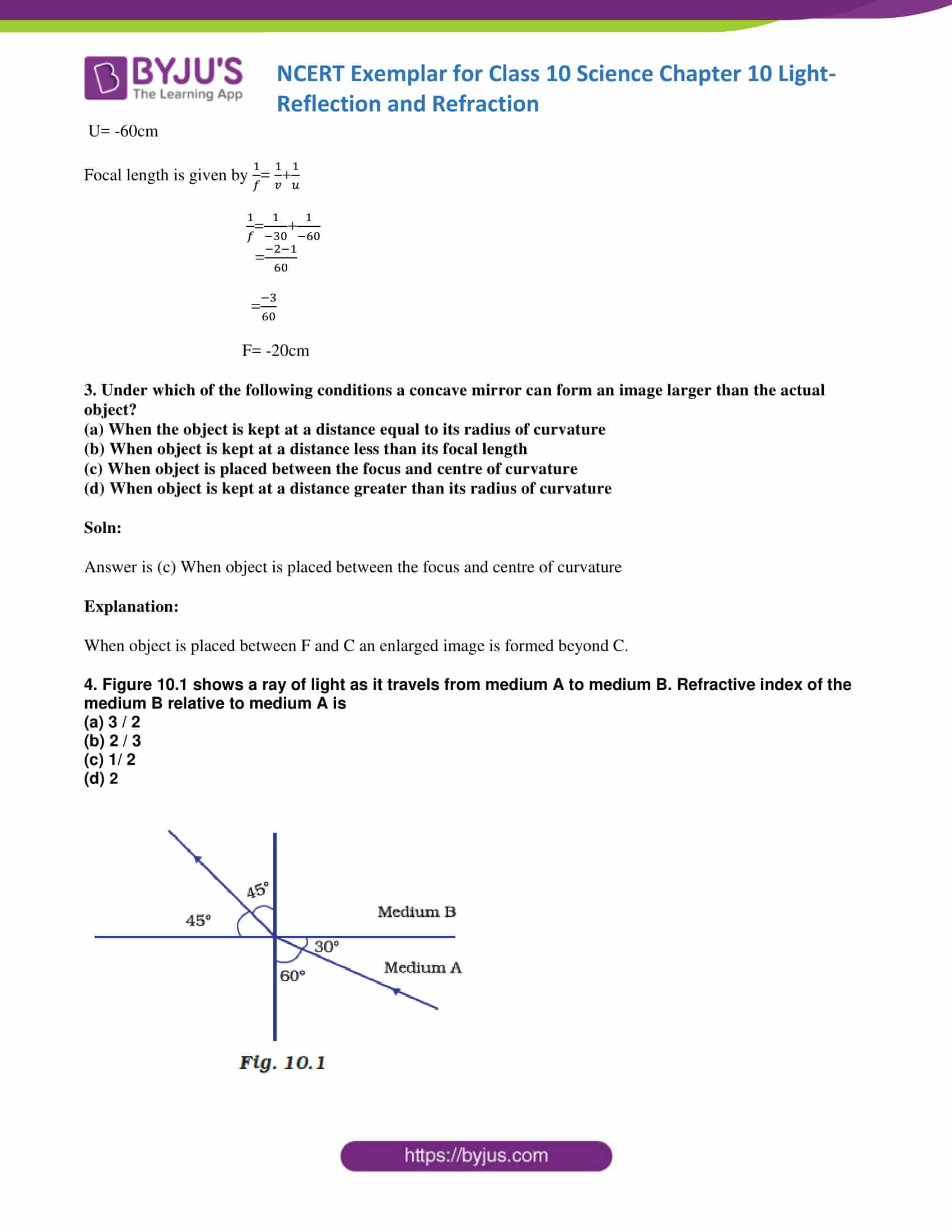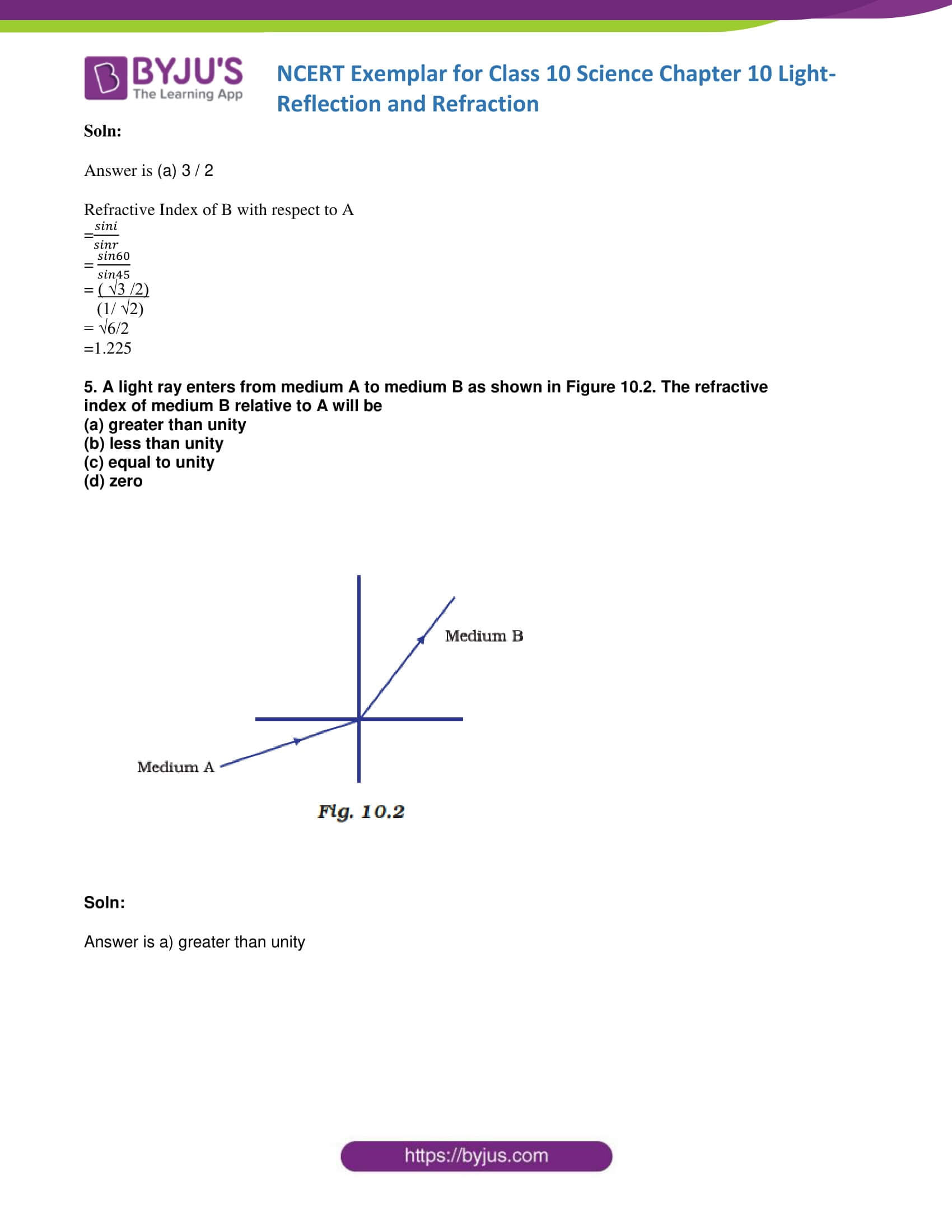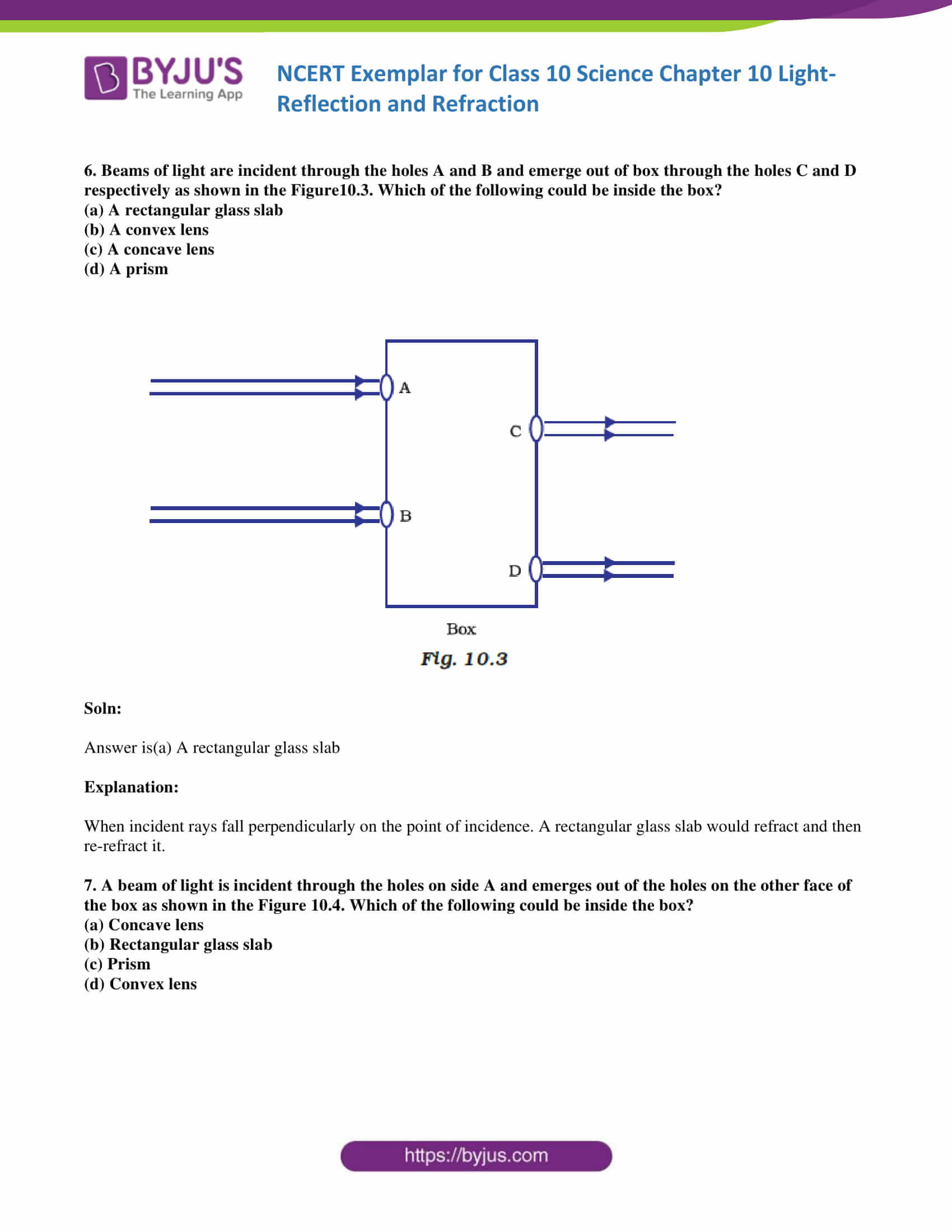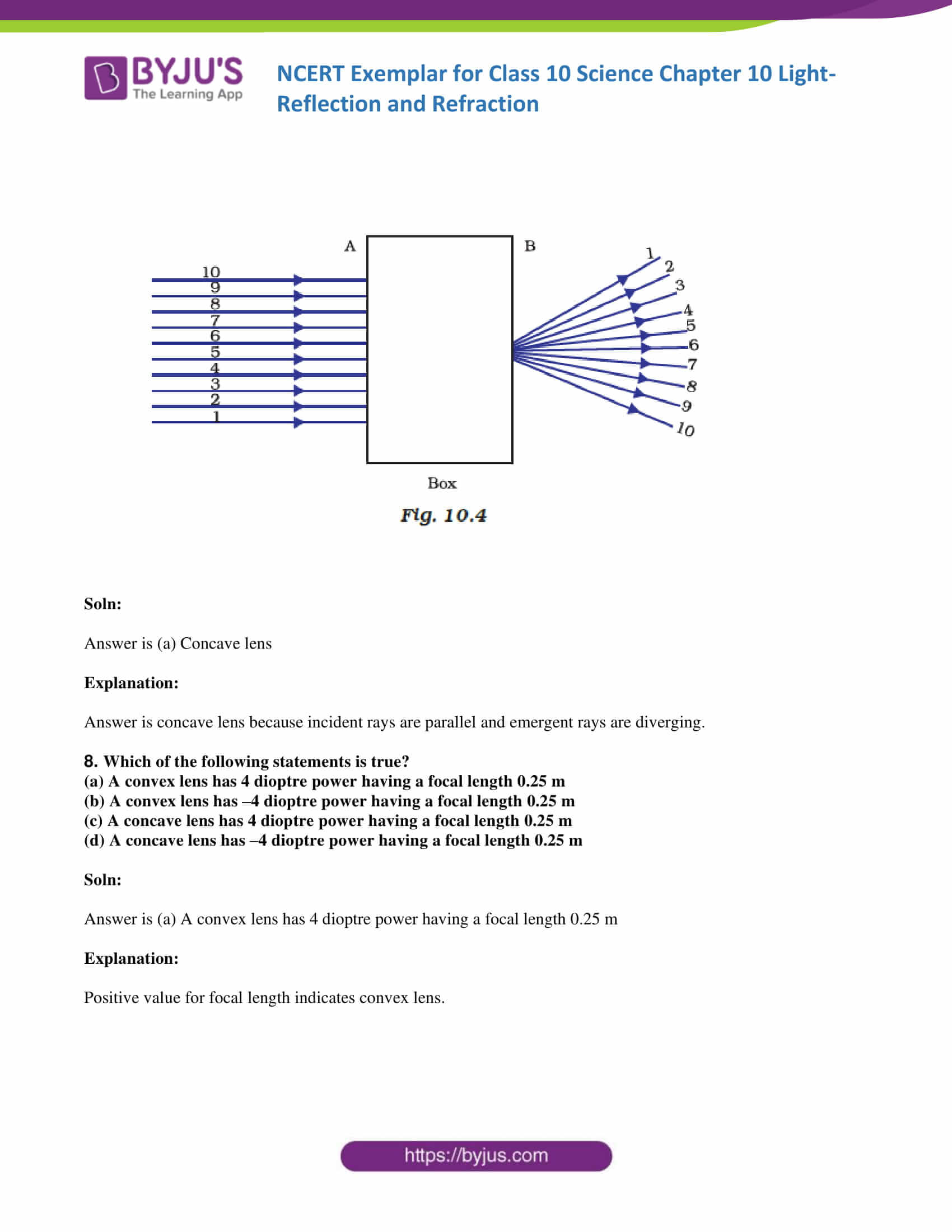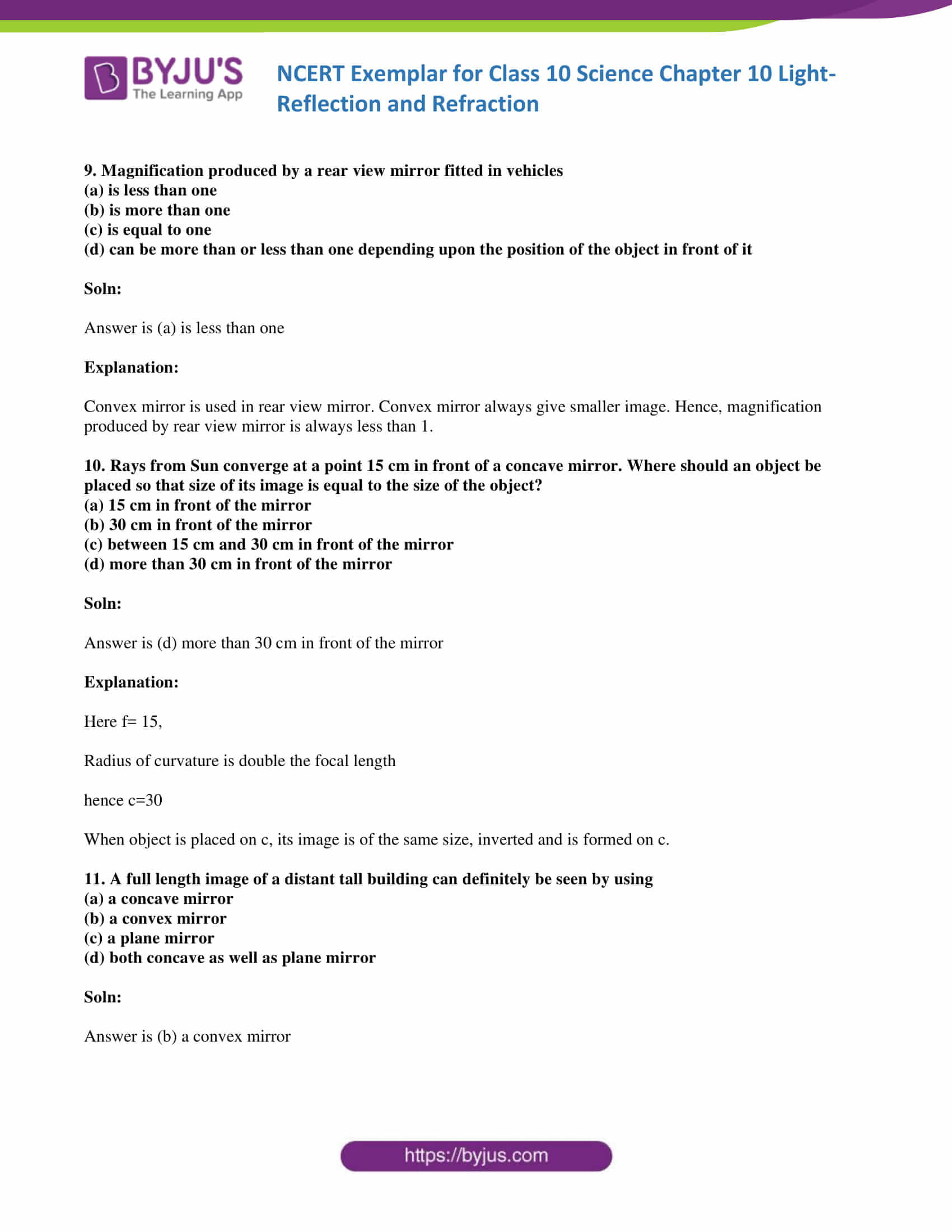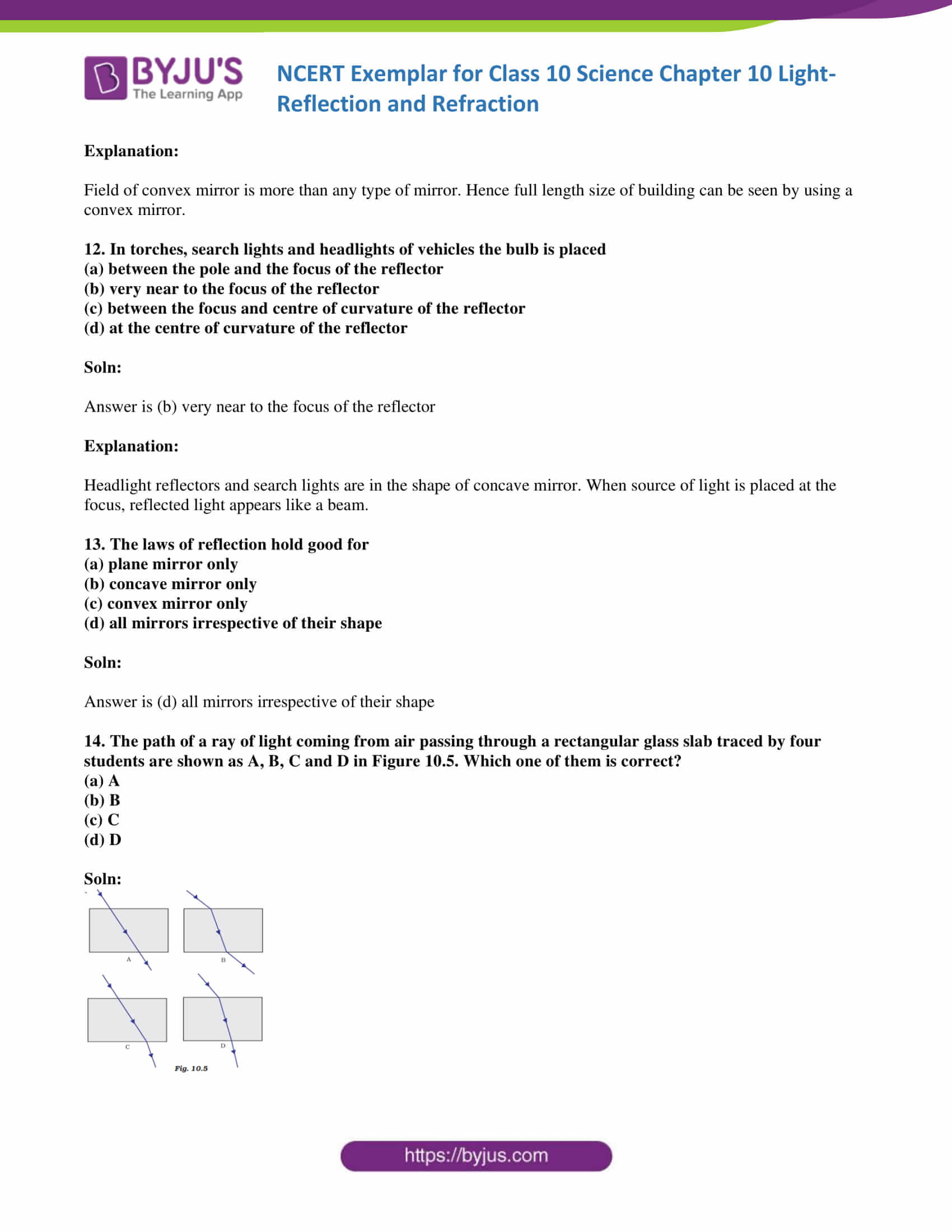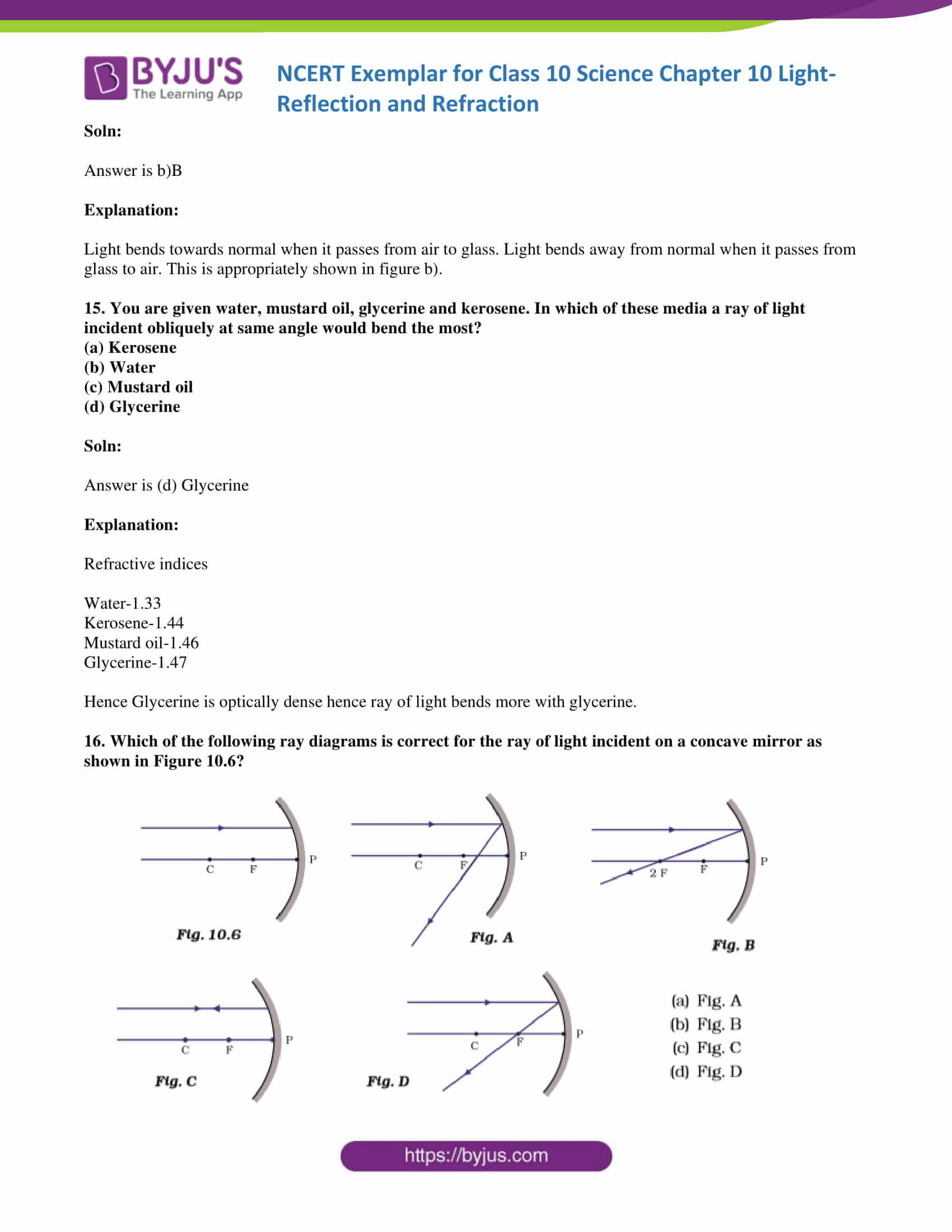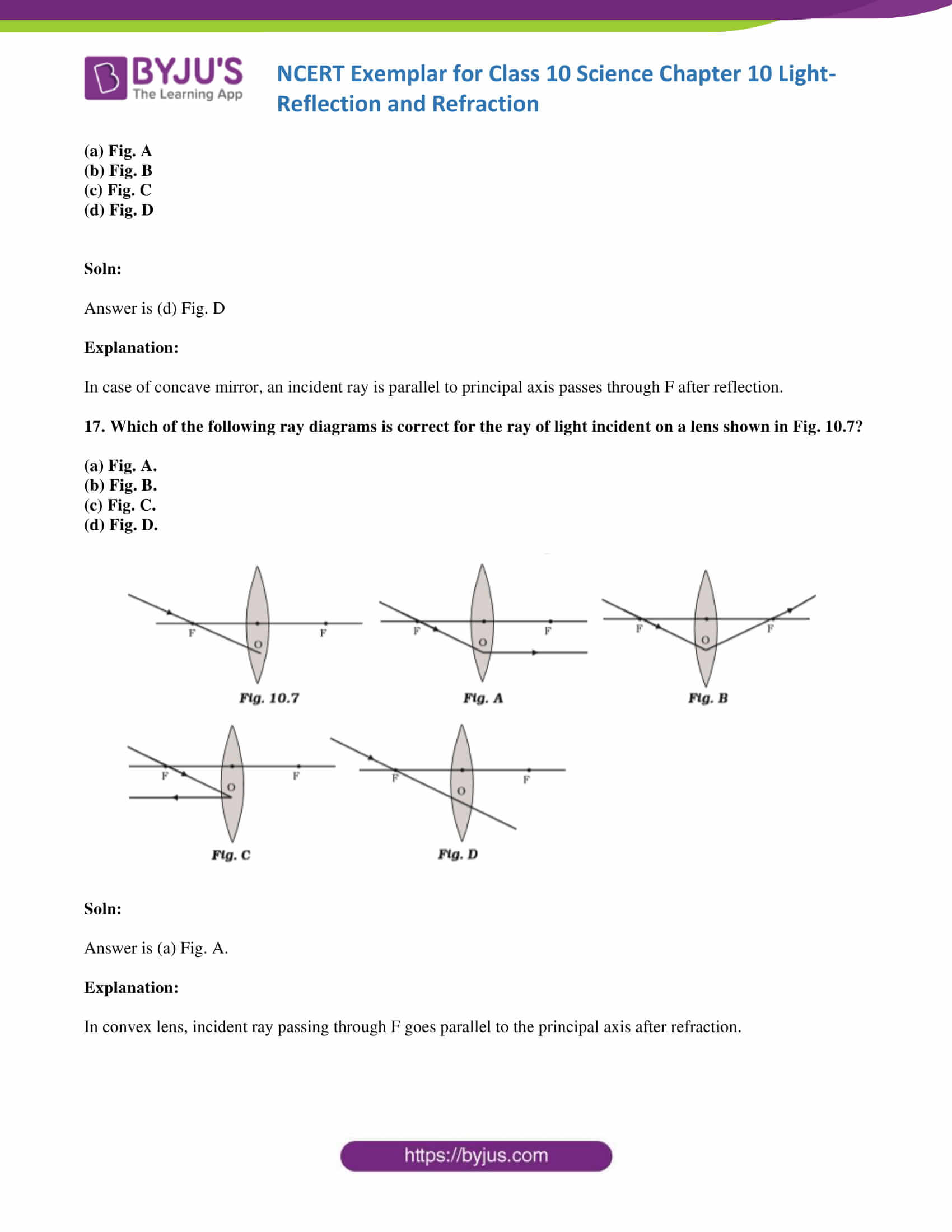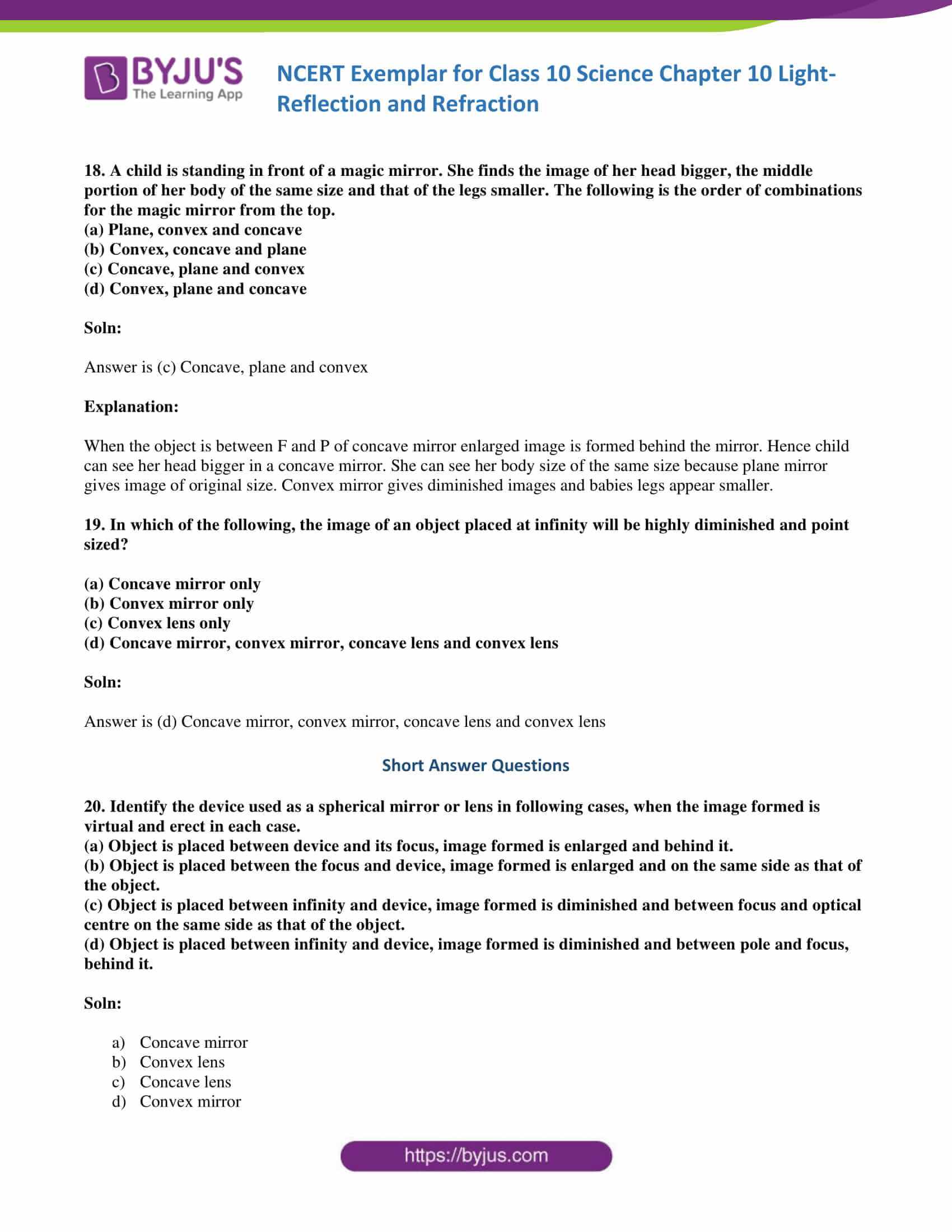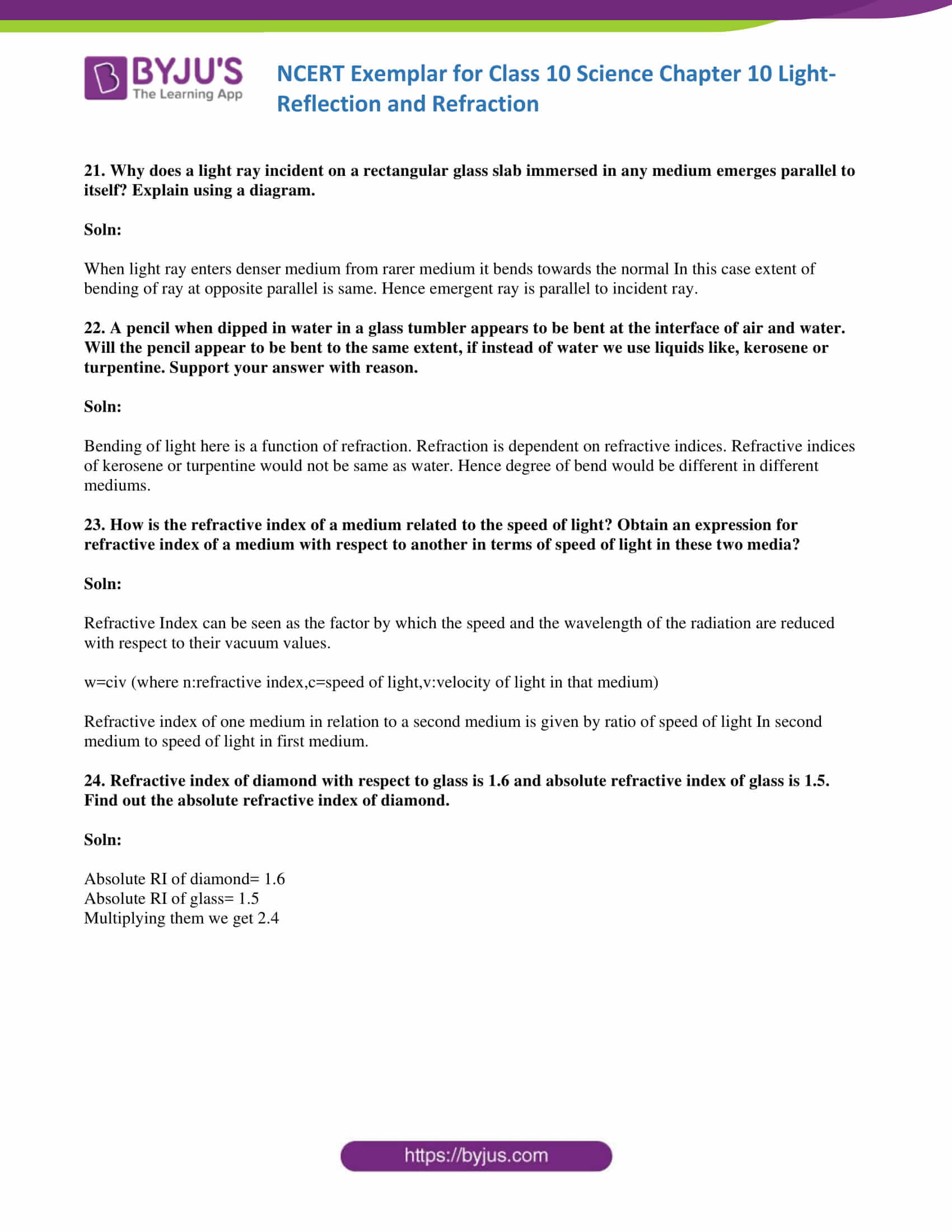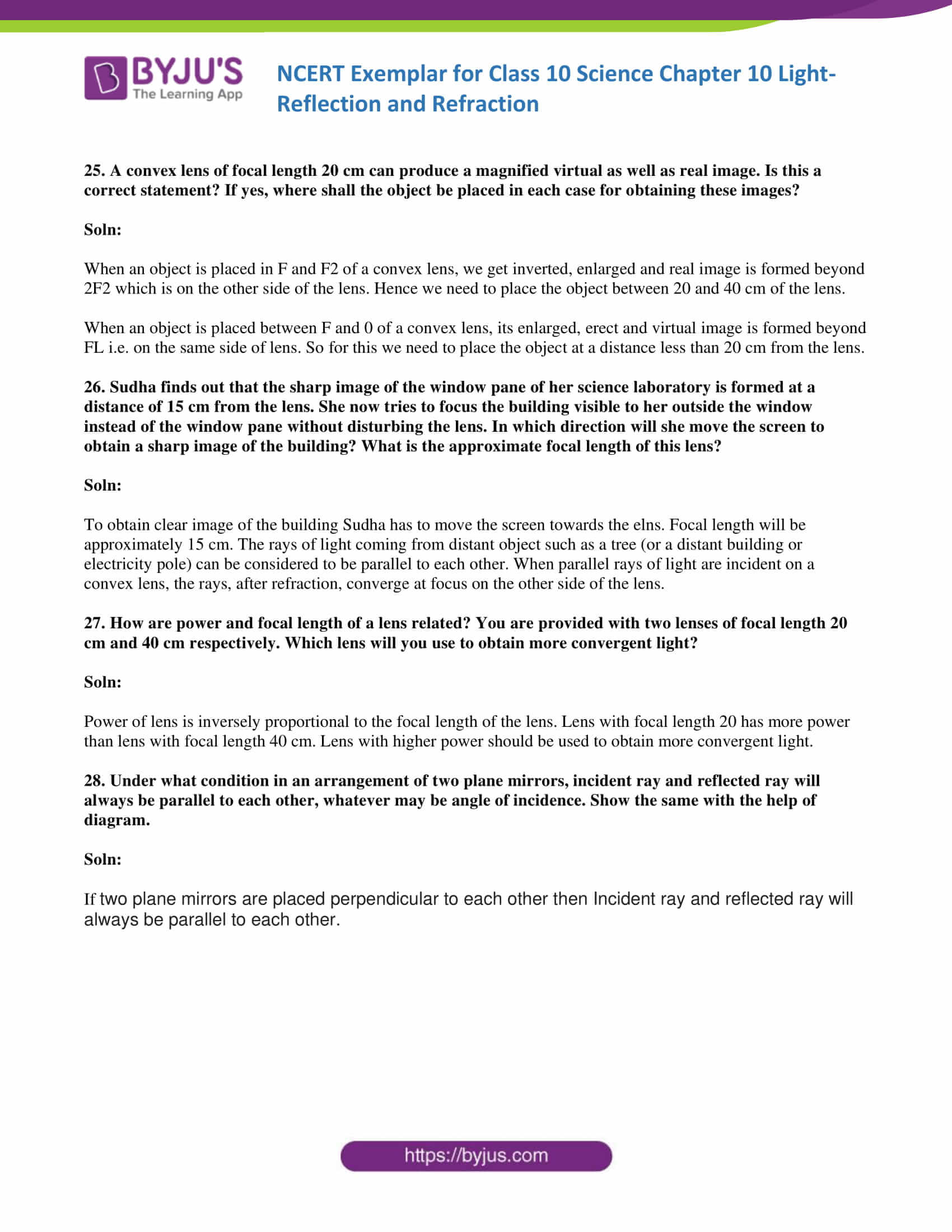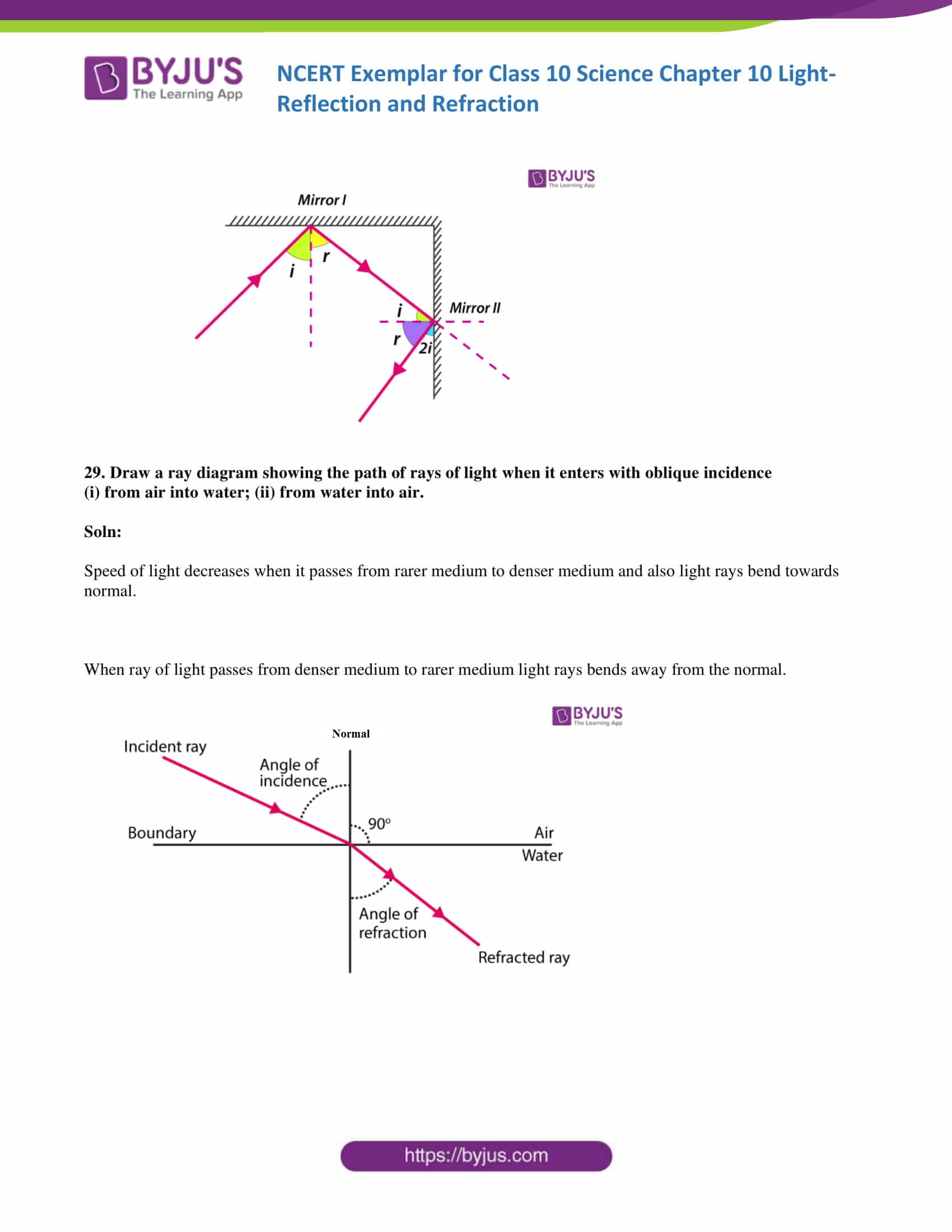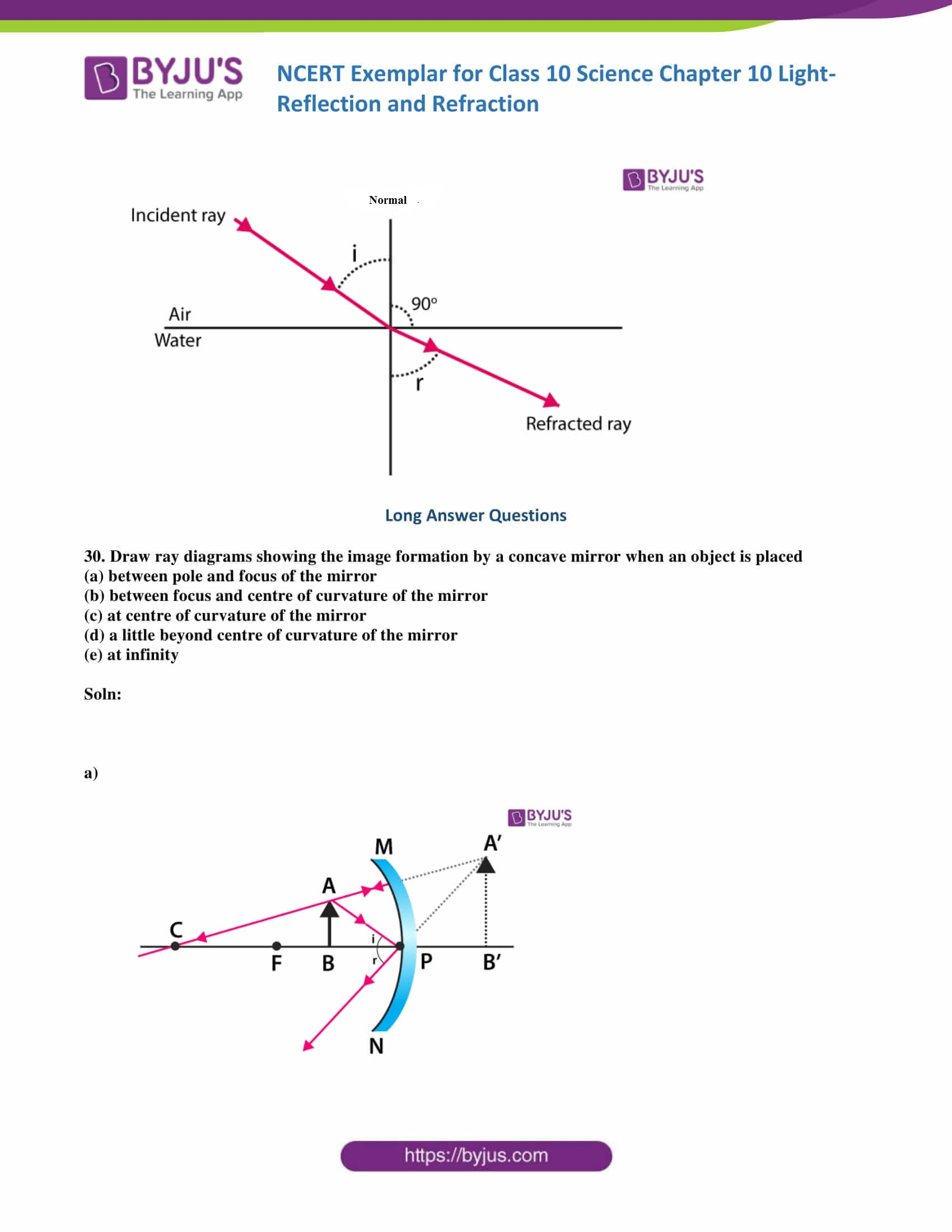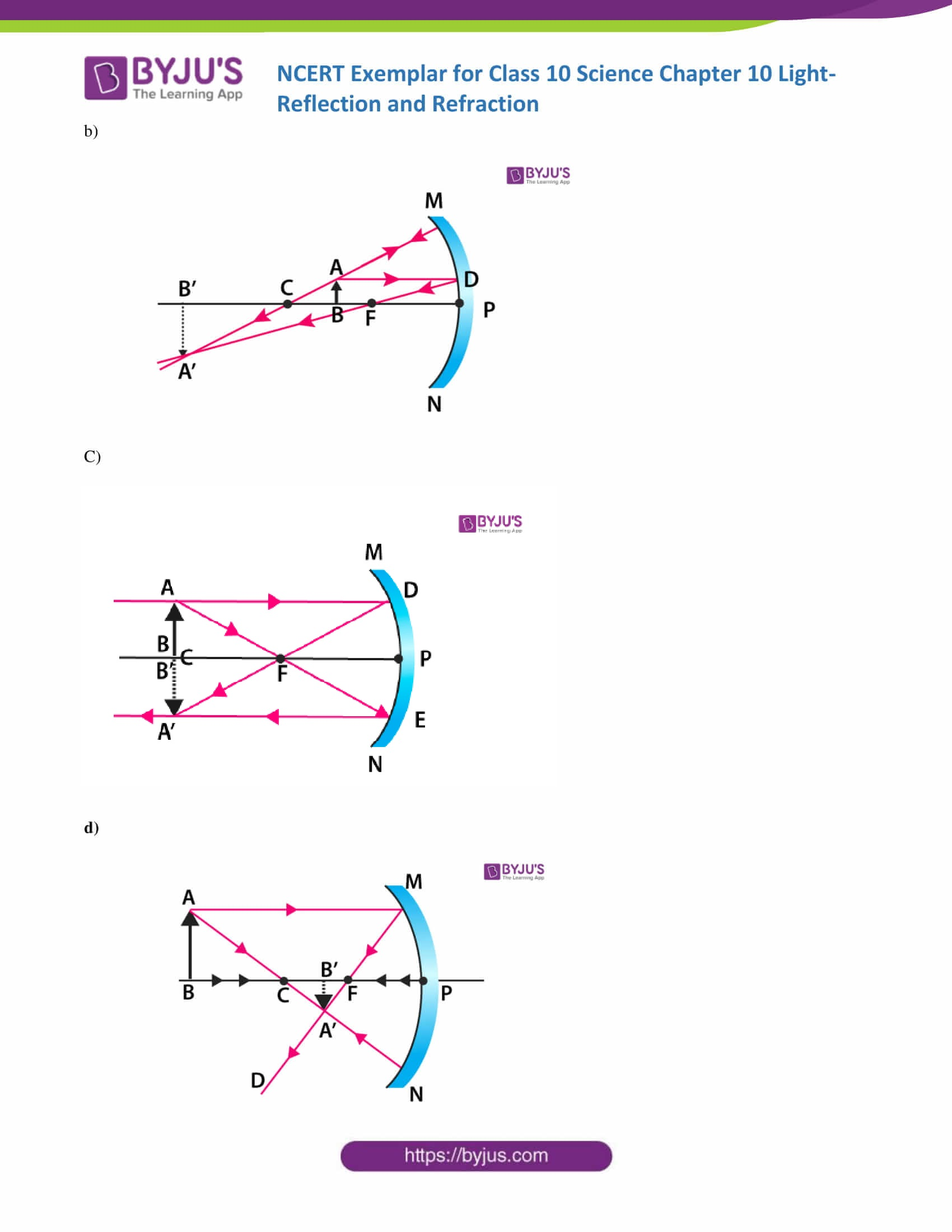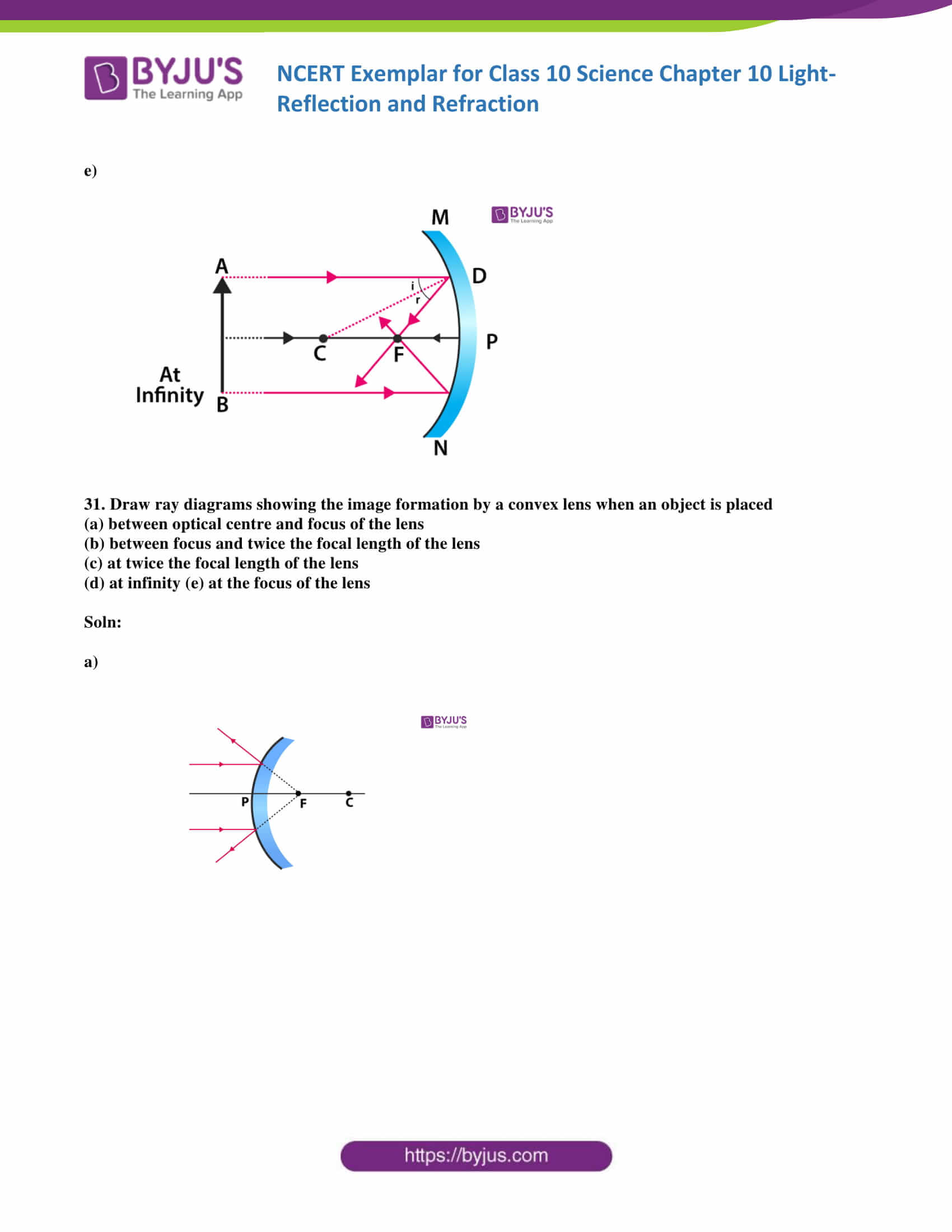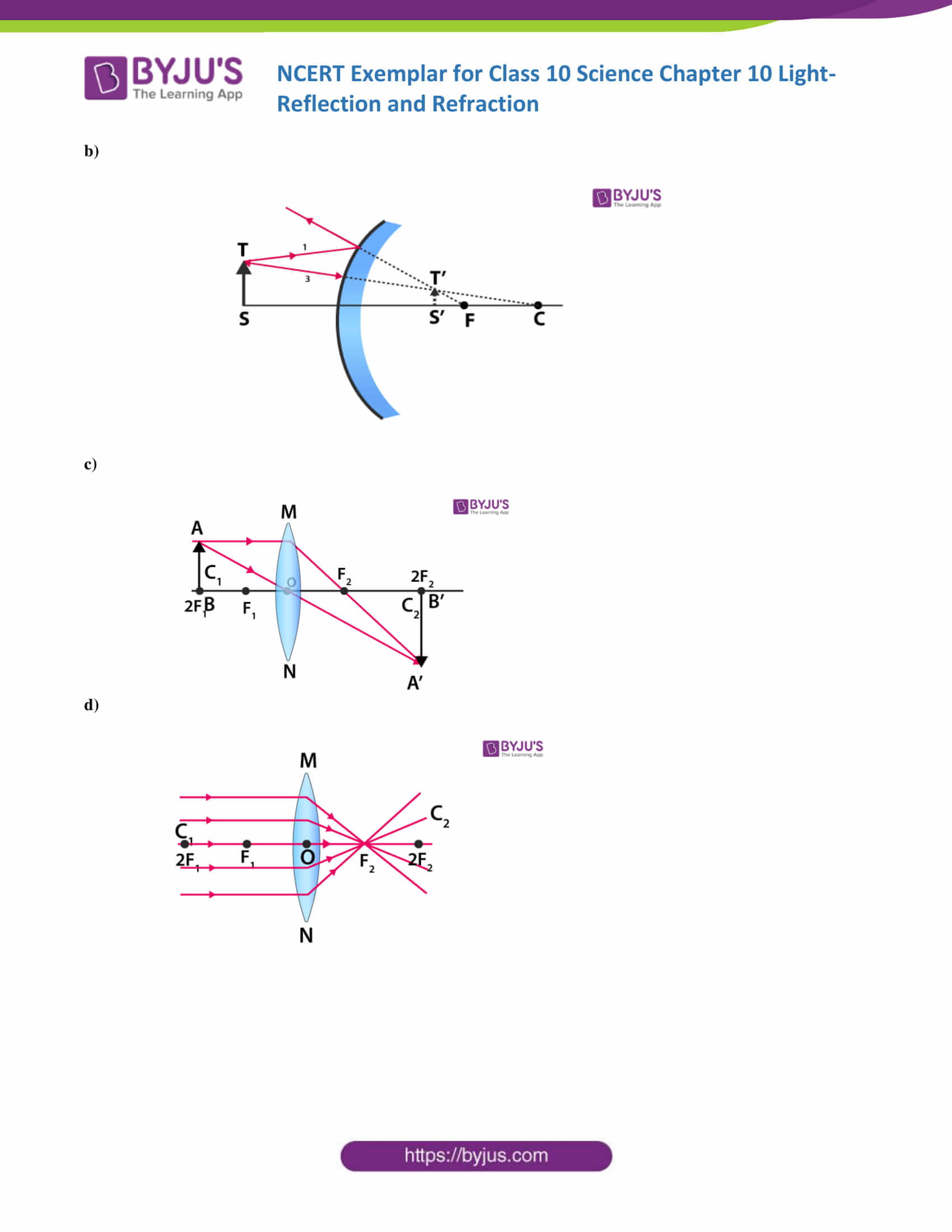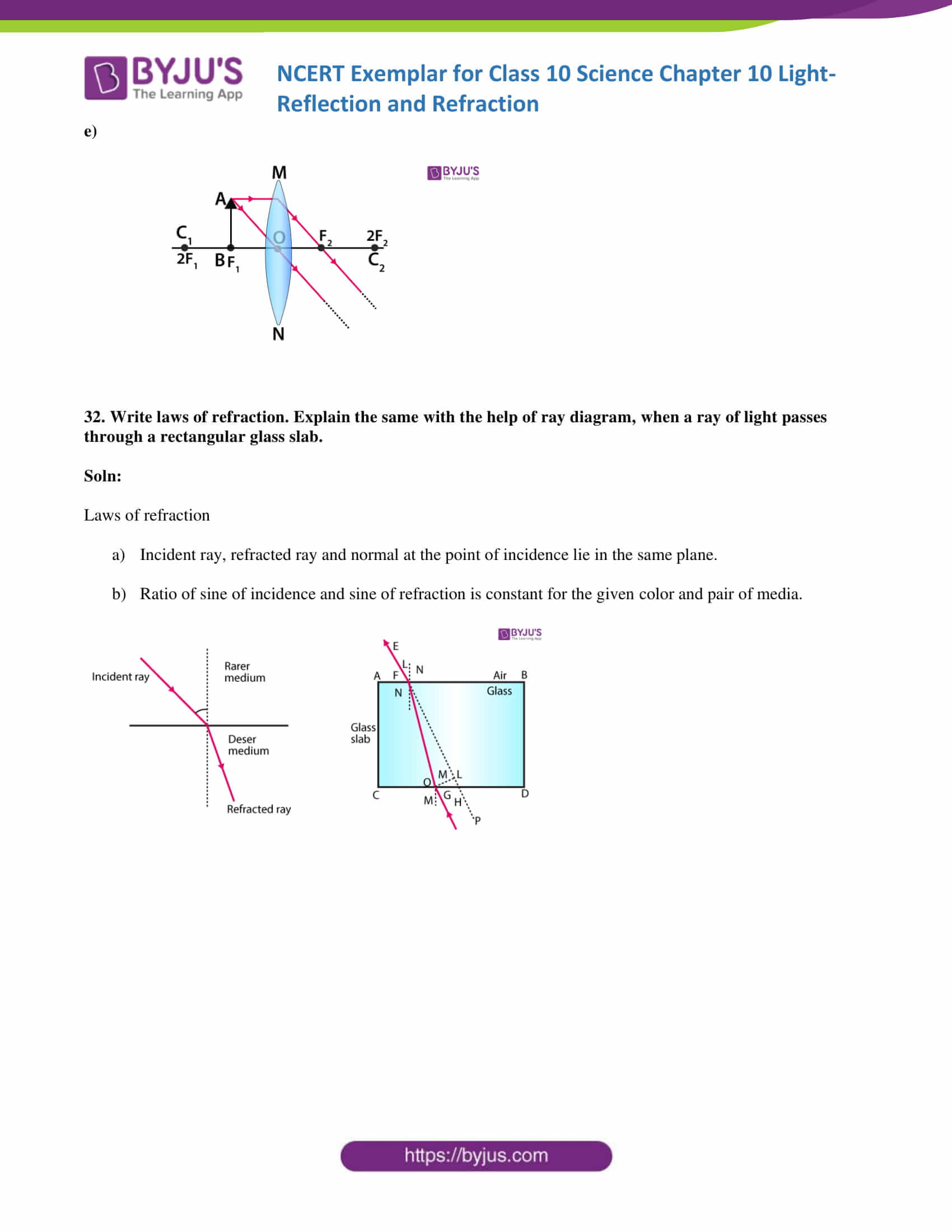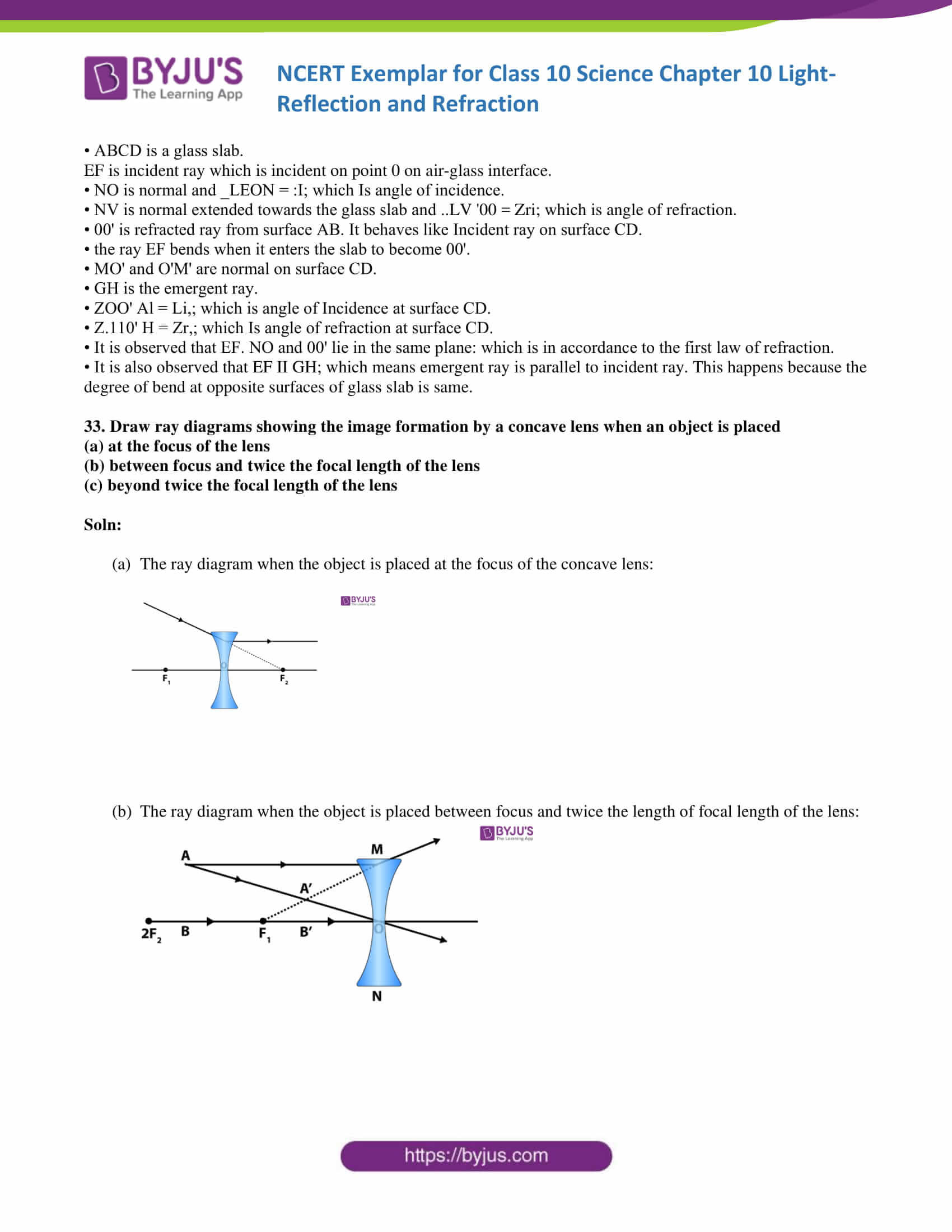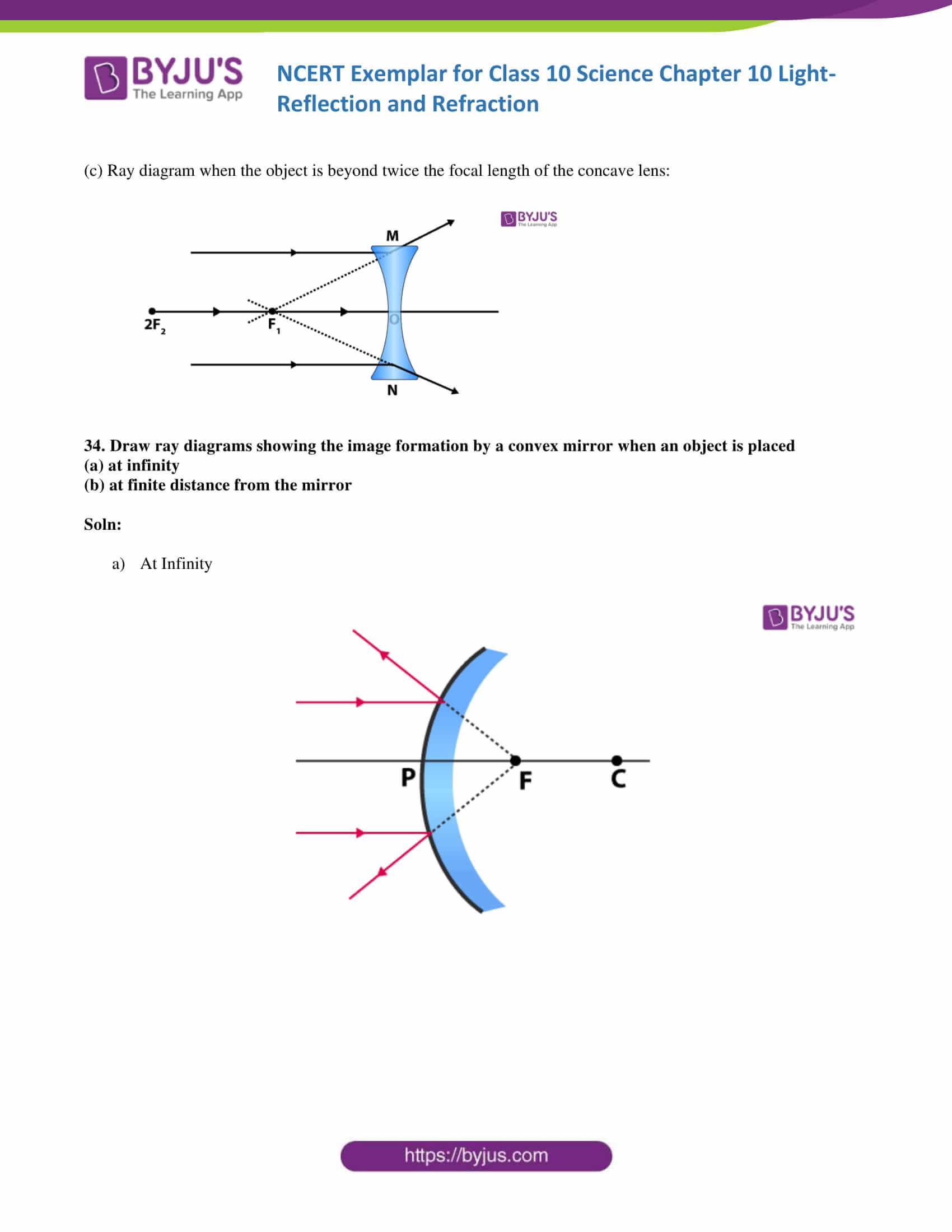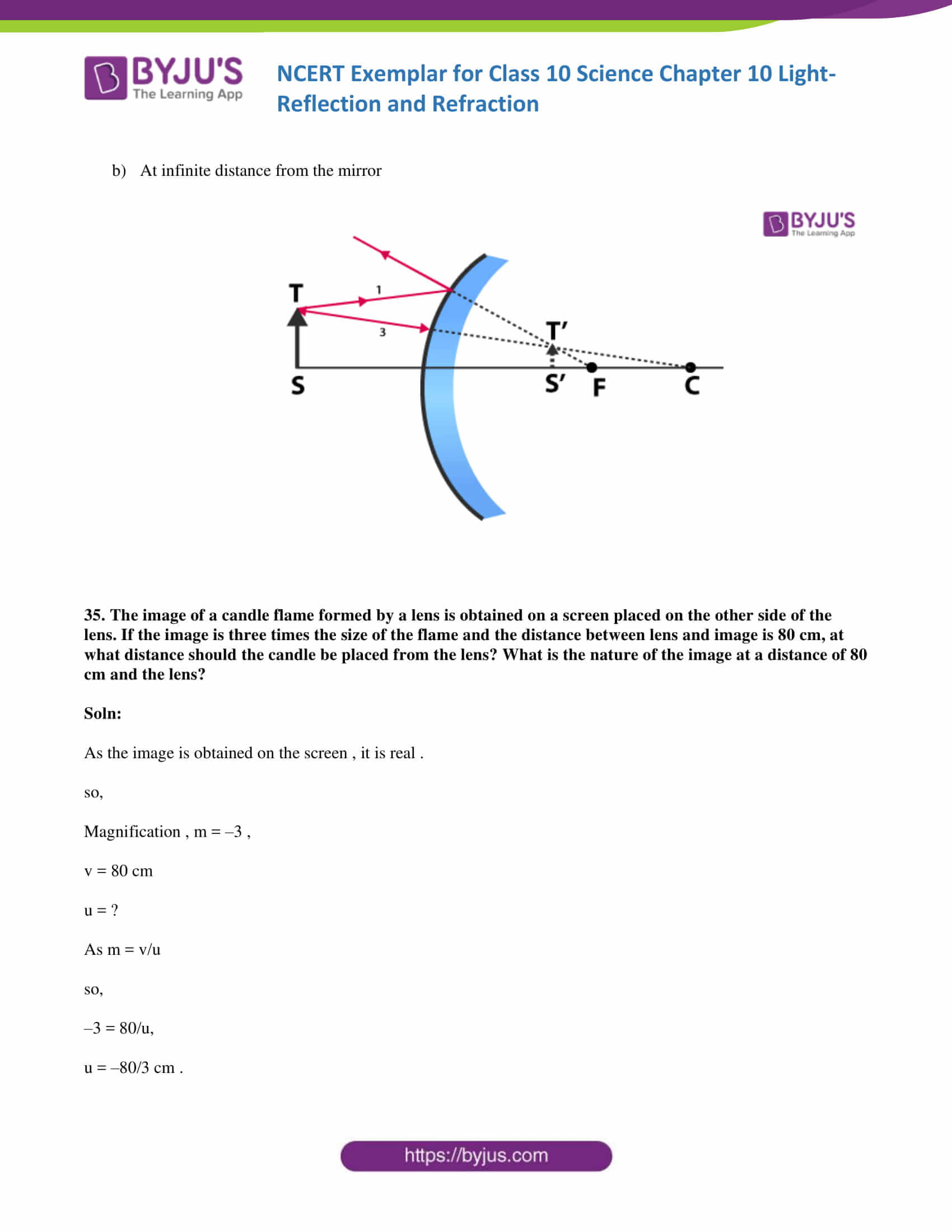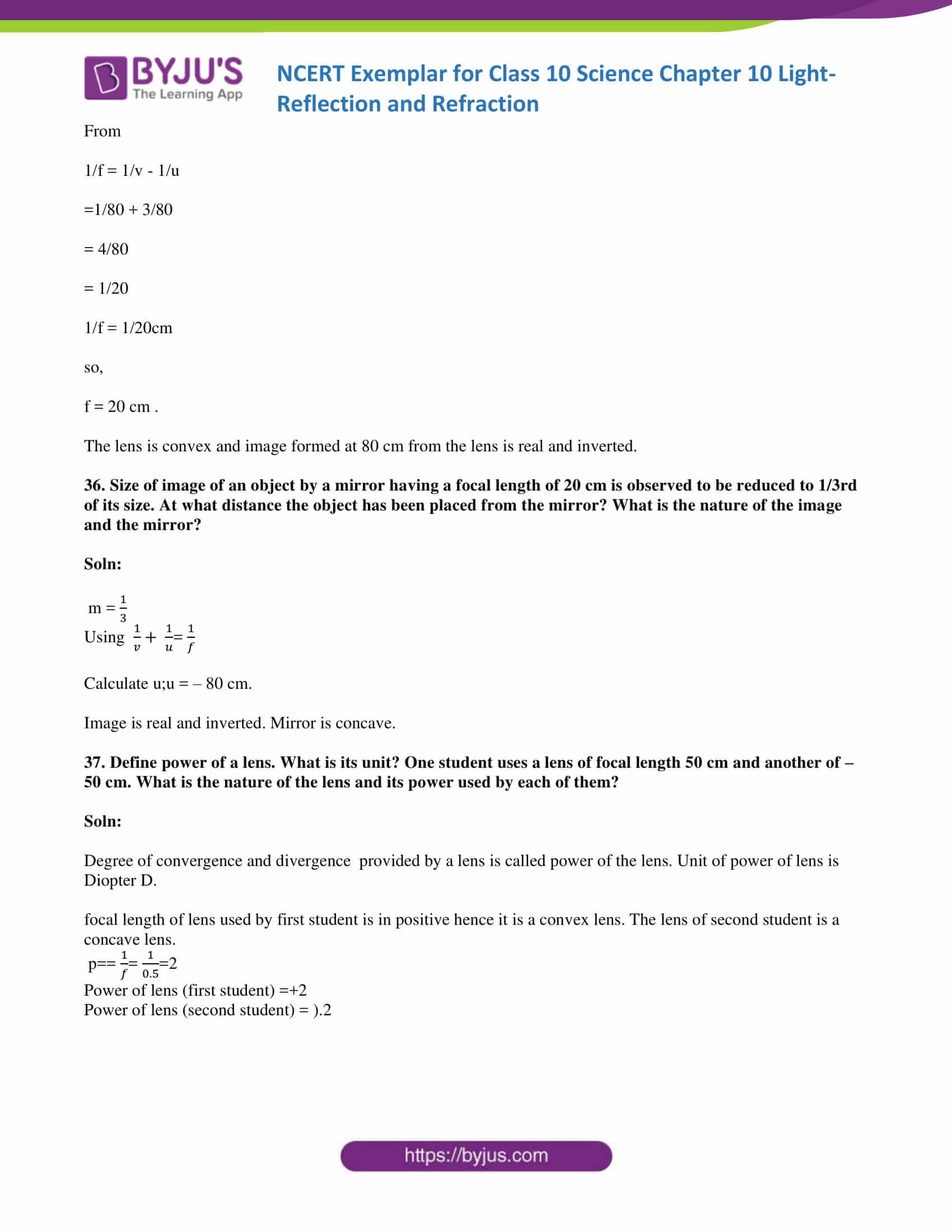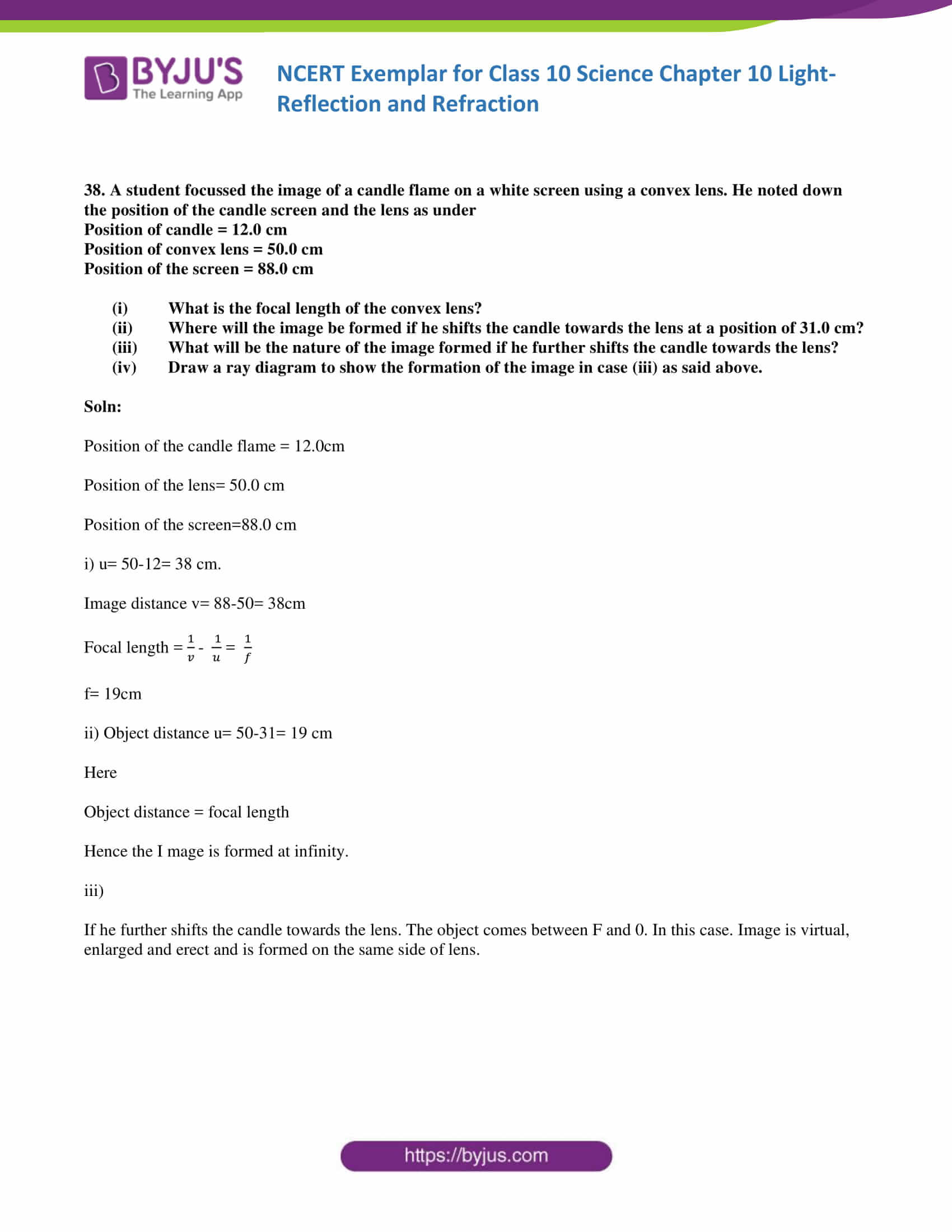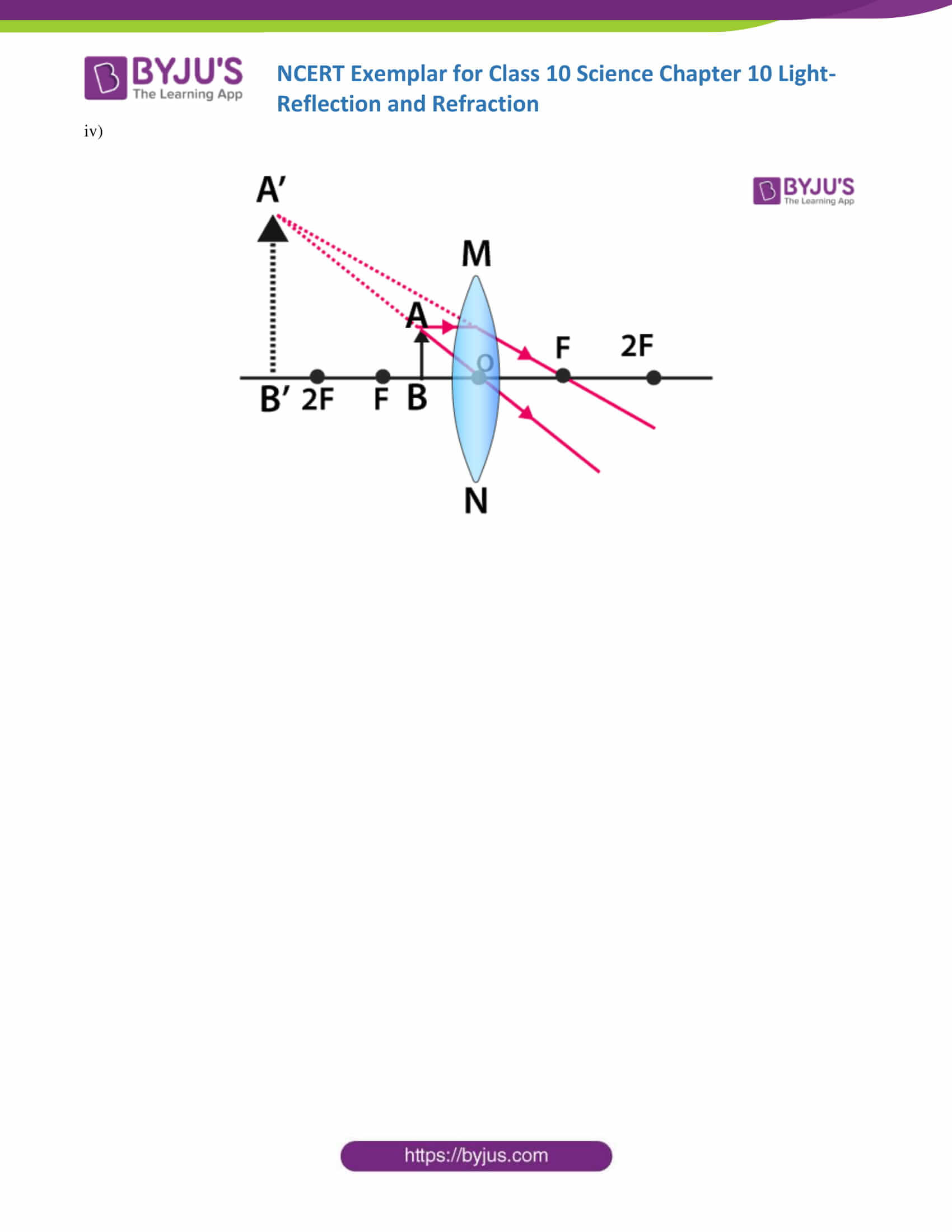### Multiple Choice Questions

1. Which of the following can make a parallel beam of light when light from a point source is incident on it?

(a) Concave mirror as well as convex lens

(b) Convex mirror as well as a concave lens

(c) Two plane mirrors placed at 90° to each other

(d) Concave mirror as well as a concave lens

Soln:

The answer is (d) Concave mirror as well as a concave lens

Explanation:

When the point source of light is focused to a convex or concave mirror emergent rays make a parallel beam of light.

2. A 10 mm long awl pin is placed vertically in front of a concave mirror. A 5 mm long image of the awl pin is formed at 30 cm in front of the mirror. The focal length of this mirror is

(a) – 30 cm

(b) – 20 cm

(c) – 40 cm

(d) – 60 cm

Soln:

Answer is (b) – 20 cm

Explanation:

Here, size of object = O = + 10.0 mm = + 1.0 cm (as, 1 cm = 10 mm)

Size of Image size = I = 5.0 mm = 0.5 cm

Image distance, v = − 30 cm   (as image is real)

Let, object distance = u

Focal length, f =?

Magnification m= I(size of image)/O(size of image)

Magnification is given by m= -v/u

i/o=-V/u

0.5/1=-30/u

U = -60cm

Focal length is given by 1/f =1/v + 1/u

1/f=1/-30 + 1/60

= -2-1/60

=-3/60

F= -20cm

3. Under which of the following conditions a concave mirror can form an image larger than the actual object?

(a) When the object is kept at a distance equal to its radius of curvature

(b) When an object is kept at a distance less than its focal length

(c) When an object is placed between the focus and centre of curvature

(d) When an object is kept at a distance greater than its radius of curvature

Soln:

Answer is (c) When an object is placed between the focus and centre of curvature

Explanation:

When an object is placed between F and C an enlarged image is formed beyond C.

4. Figure 10.1 shows a ray of light as it travels from medium A to medium B. Refractive index of the

medium B relative to medium A is

(a) √3 / √2

(b) 2 / 3

(c) 1/ 2

(d) 2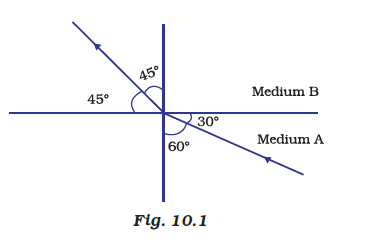Soln:

Answer is (a) 3 / 2

Refractive Index of B with respect to A

=sin i/sin r

=sin60/sin45

= ( √3 /2) /(1/ √2)

= √3 / √2

5. A light ray enters from medium A to medium B as shown in Figure 10.2. The refractive

index of medium B relative to A will be

(a) greater than unity

(b) less than unity

(c) equal to unity

(d) zero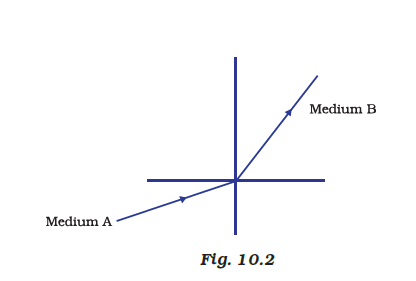Soln:

The answer is (a) greater than unity

6. Beams of light are incident through the holes A and B and emerge out of the box through the holes C and D respectively as shown in the Figure10.3. Which of the following could be inside the box?

(a) A rectangular glass slab

(b) A convex lens

(c) A concave lens

(d) A prism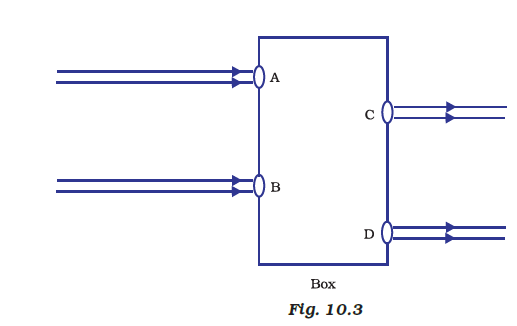Soln:

Answer is(a) A rectangular glass slab

Explanation:

When incident rays fall perpendicularly on the point of incidence. A rectangular glass slab would refract and then re-refract it.

7. A beam of light is incident through the holes on side A and emerges out of the holes on the other face of the box as shown in Figure 10.4. Which of the following could be inside the box?

(a) Concave lens

(b) Rectangular glass slab

(c) Prism

(d) Convex lens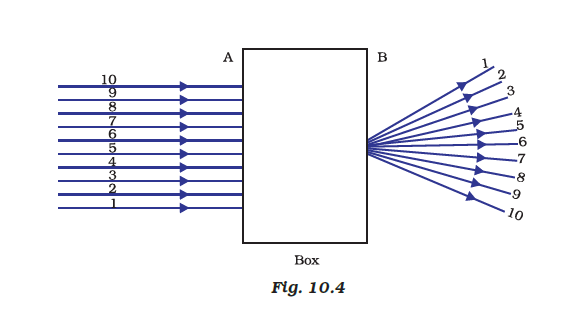Soln:

Explanation:

Convex lenses are converging lenses. They have the ability to converge a parallel beam of light into a point.

8. Which of the following statements is true?

(a) A convex lens has 4 dioptre power having a focal length 0.25 m

(b) A convex lens has –4 dioptre power having a focal length 0.25 m

(c) A concave lens has 4 dioptre power having a focal length 0.25 m

(d) A concave lens has –4 dioptre power having a focal length 0.25 m

Soln:

Answer is (a) A convex lens has 4 dioptre power having a focal length 0.25 m

Explanation:

Positive value for focal length indicates a convex lens.

9. Magnification produced by a rearview mirror fitted in vehicles

(a) is less than one

(b) is more than one

(c) is equal to one

(d) can be more than or less than one depending upon the position of the object in front of it

Soln:

Answer is (a) is less than one

Explanation:

Convex mirror is used in the rearview mirror. Convex mirror always give a smaller image. Hence, magnification produced by rear view mirror is always less than 1.

10. Rays from Sun converge at a point 15 cm in front of a concave mirror. Where should an object be placed so that size of its image is equal to the size of the object?

(a) 15 cm in front of the mirror

(b) 30 cm in front of the mirror

(c) between 15 cm and 30 cm in front of the mirror

(d) more than 30 cm in front of the mirror

Soln:

The answer is (d) more than 30 cm in front of the mirror

Explanation:

Here f= 15,

Radius of curvature is double the focal length

hence c=30

When an object is placed on c, its image is of the same size, inverted and is formed on c.

11. A full-length image of a distant tall building can definitely be seen by using

(a) a concave mirror

(b) a convex mirror

(c) a plane mirror

(d) both concave as well as plane mirror

Soln:

The answer is (b) a convex mirror

Explanation:

Field of convex mirror is more than any type of mirror. Hence full-length size of building can be seen by using a convex mirror.

12. In torches, searchlights and headlights of vehicles the bulb is placed

(a) between the pole and the focus of the reflector

(b) very near to the focus of the reflector

(c) between the focus and centre of curvature of the reflector

(d) at the centre of curvature of the reflector

Soln:

The answer is (b) very near to the focus of the reflector

Explanation:

Headlight reflectors and searchlights are in the shape of concave mirror. When the source of light is placed at the focus, reflected light appears like a beam.

13. The laws of reflection hold good for

(a) plane mirror only

(b) concave mirror only

(c) convex mirror only

(d) all mirrors irrespective of their shape

Soln:

The answer is (d) all mirrors irrespective of their shape

14. The path of a ray of light coming from air passing through a rectangular glass slab traced by four students are shown as A, B, C and D in Figure 10.5. Which one of them is correct?

(a) A

(b) B

(c) C

(d) D

Soln: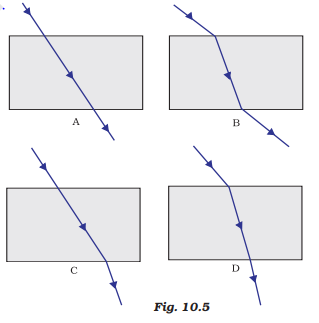Soln:

Explanation:

Light bends towards normal when it passes from air to glass. Light bends away from normal when it passes from glass to air. This is appropriately shown in figure b).

15. You are given water, mustard oil, glycerine and kerosene. In which of these media a ray of light incident obliquely at same angle would bend the most?

(a) Kerosene

(b) Water

(c) Mustard oil

(d) Glycerine

Soln:

Explanation:

Refractive indices

Water-1.33

Kerosene-1.44

Mustard oil-1.46

Glycerine-1.47

Hence Glycerine is optically dense hence ray of light bends more with glycerine.

16. Which of the following ray diagrams is correct for the ray of light incident on a concave mirror as shown in Figure 10.6?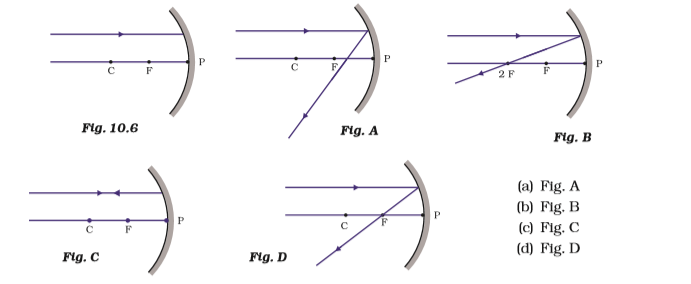(a) Fig. A

(b) Fig. B

(c) Fig. C

(d) Fig. D

Soln:

The answer is (d) Fig. D

Explanation:

In case of concave mirror, an incident ray is parallel to the principal axis passes through F after reflection.

17. Which of the following ray diagrams is correct for the ray of light incident on a lens shown in Fig. 10.7?

(a) Fig. A.

(b) Fig. B.

(c) Fig. C.

(d) Fig. D.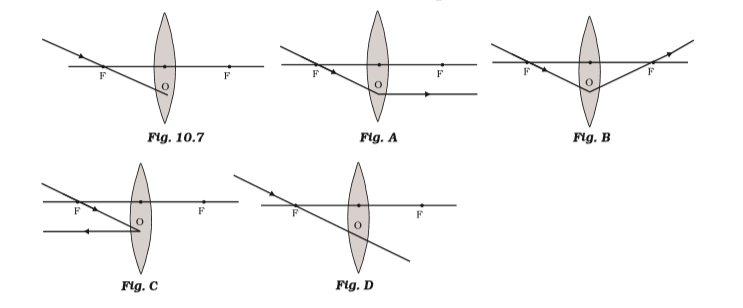Soln:

Explanation:

In convex lens, incident ray passing through F goes parallel to the principal axis after refraction.

18. A child is standing in front of a magic mirror. She finds the image of her head bigger, the middle portion of her body of the same size and that of the legs smaller. The following is the order of combinations for the magic mirror from the top.

(a) Plane, convex and concave

(b) Convex, concave and plane

(c) Concave, plane and convex

(d) Convex, plane and concave

Soln:

Answer is (c) Concave, plane and convex

Explanation:

When the object is between F and P of concave mirror enlarged image is formed behind the mirror. Hence child can see her head bigger in a concave mirror. She can see her body size of the same size because the plane mirror gives an image of the original size. Convex mirror gives diminished images and babies legs appear smaller.

19. In which of the following, the image of an object placed at infinity will be highly diminished and point sized?

(a) Concave mirror only

(b) Convex mirror only

(c) Convex lens only

(d) Concave mirror, convex mirror, concave lens and convex lens

Soln:

The answer is (d) Concave mirror, convex mirror, concave lens and convex lens

20. Identify the device used as a spherical mirror or lens in the following cases, when the image formed is virtual and erect in each case.

(a) Object is placed between the device and its focus, an image formed is enlarged and behind it.

(b) Object is placed between the focus and device, an image formed is enlarged and on the same side as that of the object.

(c) Object is placed between infinity and device, an image formed is diminished and between focus and optical centre on the same side as that of the object.

(d) Object is placed between infinity and device, image formed is diminished and between pole and focus, behind it.

Soln:

1. Concave mirror
2. Convex lens
3. Concave lens
4. Convex mirror

21. Why does a light ray incident on a rectangular glass slab immersed in any medium emerges parallel to itself? Explain using a diagram.

Soln:

When light ray enters denser medium from the rarer medium it bends towards the normal In this case extent of bending of the ray at the opposite parallel is the same. Hence emergent ray is parallel to the incident ray.

22. A pencil, when dipped in water in a glass tumbler, appears to be bent at the interface of air and water. Will the pencil appear to be bent to the same extent, if instead of water we use liquids like, kerosene or turpentine. Support your answer with reason.

Soln:

Bending of light here is a function of refraction. Refraction is dependent on refractive indices. Refractive indices of kerosene or turpentine would not be the same as water. Hence degree of bend would be different in different mediums.

23. How is the refractive index of a medium related to the speed of light? Obtain an expression for the refractive index of a medium with respect to another in terms of speed of light in these two media?

Soln:

Refractive Index can be seen as the factor by which the speed and the wavelength of the radiation are reduced with respect to their vacuum values.

w=civ (where n:refractive index,c=speed of light,v:velocity of light in that medium)

Refractive index of one medium in relation to a second medium is given by the ratio of the speed of light In the second medium to speed of light in the first medium.

24. Refractive index of diamond with respect to glass is 1.6 and the absolute refractive index of glass is 1.5. Find out the absolute refractive index of diamond.

Soln:

Absolute RI of diamond= 1.6

Absolute RI of glass= 1.5

Multiplying them we get 2.4

25. A convex lens of focal length 20 cm can produce a magnified virtual as well as real image. Is this a correct statement? If yes, where shall the object be placed in each case for obtaining these images?

Soln:

When an object is placed in F and F2 of a convex lens, we get an inverted, enlarged and real image is formed beyond 2F2 which is on the other side of the lens. Hence we need to place the object between 20 and 40 cm of the lens.

When an object is placed between F and 0 of a convex lens, its enlarged, erect and virtual image is formed beyond FL i.e. on the same side of the lens. So for this, we need to place the object at a distance less than 20 cm from the lens.

26. Sudha finds out that the sharp image of the window pane of her science laboratory is formed at a distance of 15 cm from the lens. She now tries to focus the building visible to her outside the window instead of the window pane without disturbing the lens. In which direction will she move the screen to obtain a sharp image of the building? What is the approximate focal length of this lens?

Soln:

To obtain a clear image of the building Sudha has to move the screen towards the lens. Focal length will be approximately 15 cm. The rays of light coming from a distant object such as a tree (or a distant building or electricity pole) can be considered to be parallel to each other. When parallel rays of light are incident on a convex lens, the rays, after refraction, converge at focus on the other side of the lens.

27. How are power and focal length of a lens related? You are provided with two lenses of focal length 20 cm and 40 cm respectively. Which lens will you use to obtain more convergent light?

Soln:

Power of lens is inversely proportional to the focal length of the lens. Lens with focal length 20 has more power than a lens with a focal length of 40 cm. Lens with higher power should be used to obtain more convergent light.

28. Under what condition in an arrangement of two plane mirrors, incident ray and reflected ray will always be parallel to each other, whatever may be the angle of incidence. Show the same with the help of a diagram.

Soln:

If two plane mirrors are placed perpendicular to each other then Incident ray and reflected ray will always be parallel to each other.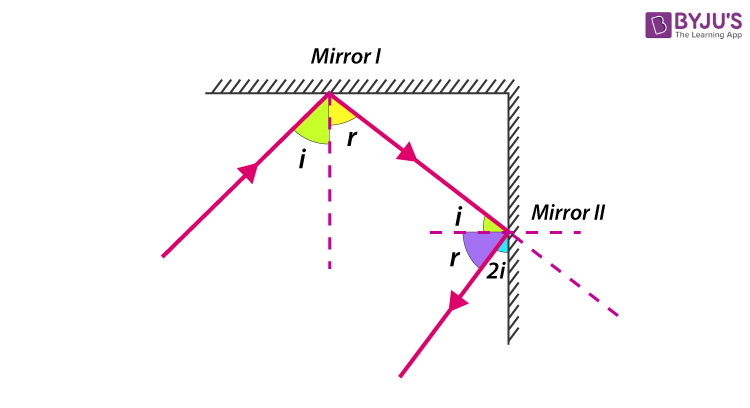29. Draw a ray diagram showing the path of rays of light when it enters with oblique incidence

(i) from air into water; (ii) from water into air.

Soln:

Speed of light decreases when it passes from rarer medium to denser medium and also light rays bend towards normal.

When a ray of light passes from a denser medium to rarer medium-light rays bends away from the normal.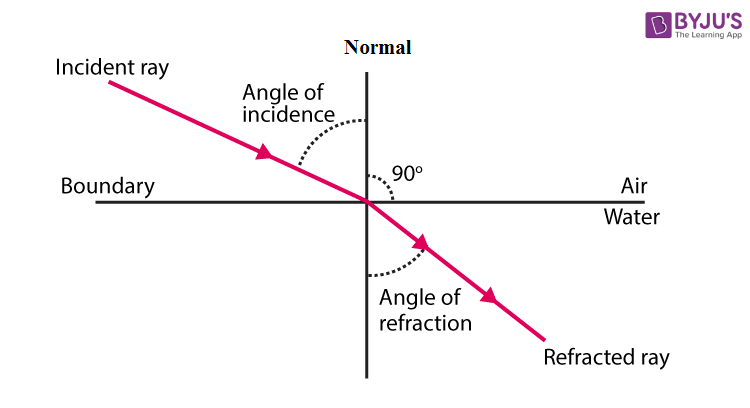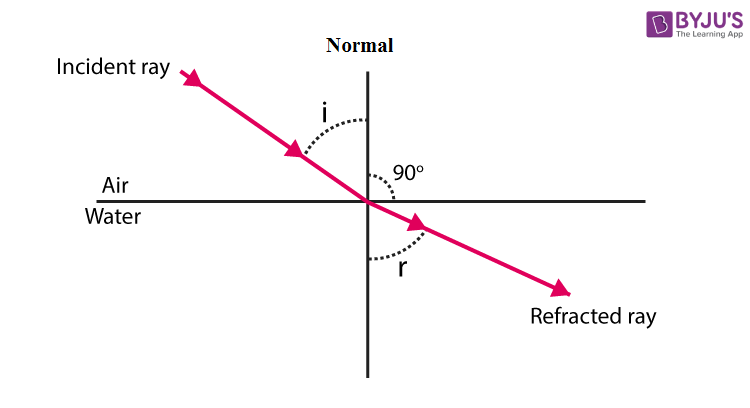30. Draw ray diagrams showing the image formation by a concave mirror when an object is placed

(a) between pole and focus of the mirror

(b) between focus and centre of curvature of the mirror

(c) at the centre of curvature of the mirror

(d) a little beyond the centre of curvature of the mirror

(e) at infinity

Soln:

a)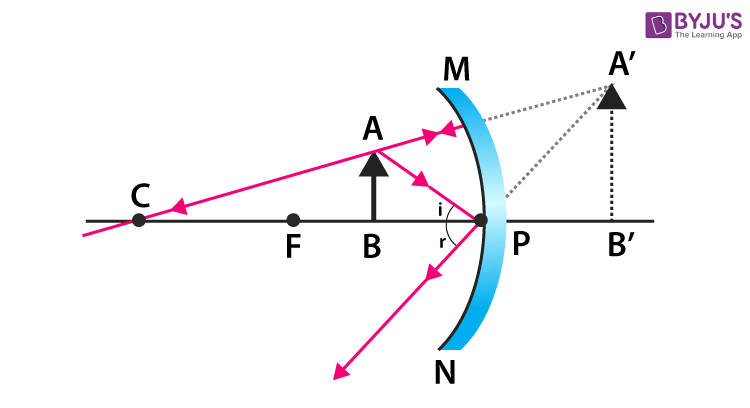b)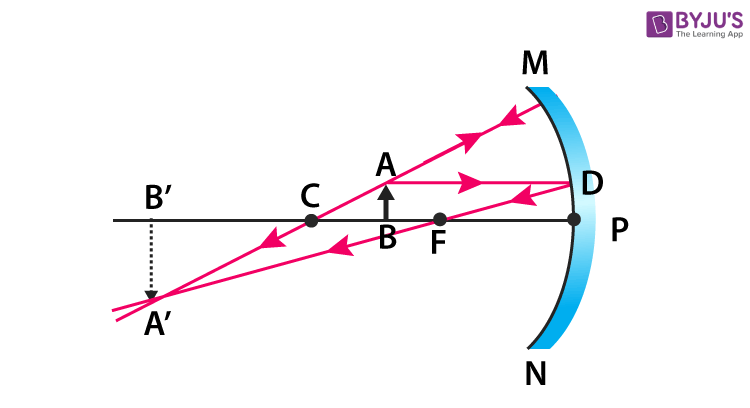C)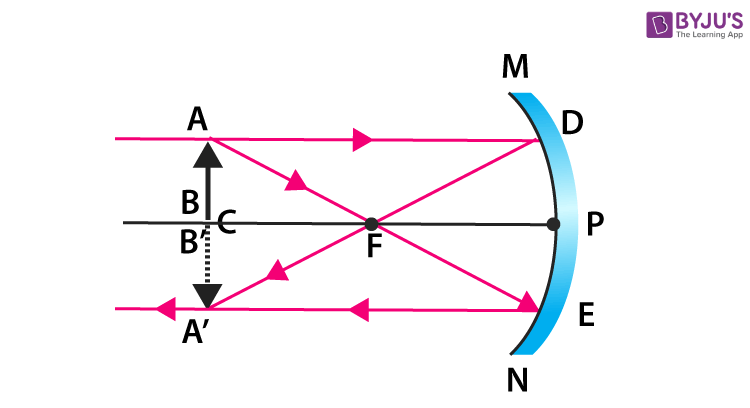d)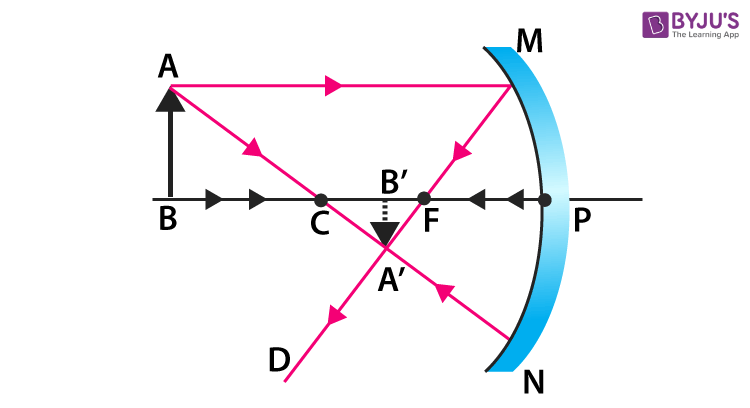e)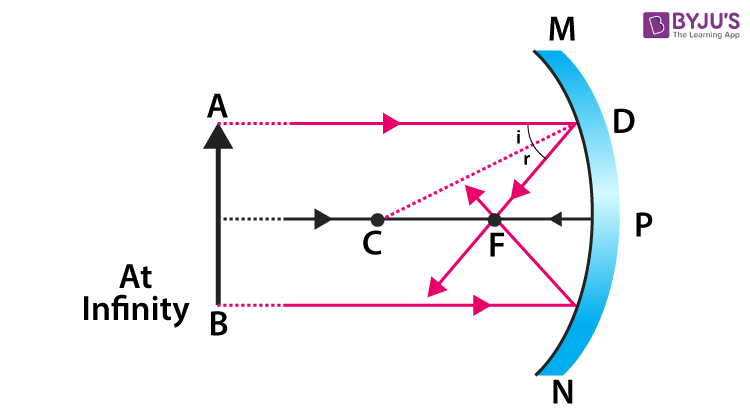31. Draw ray diagrams showing the image formation by a convex lens when an object is placed

(a) between optical centre and focus of the lens

(b) between focus and twice the focal length of the lens

(c) at twice the focal length of the lens

(d) at infinity (e) at the focus of the lens

Soln:

a)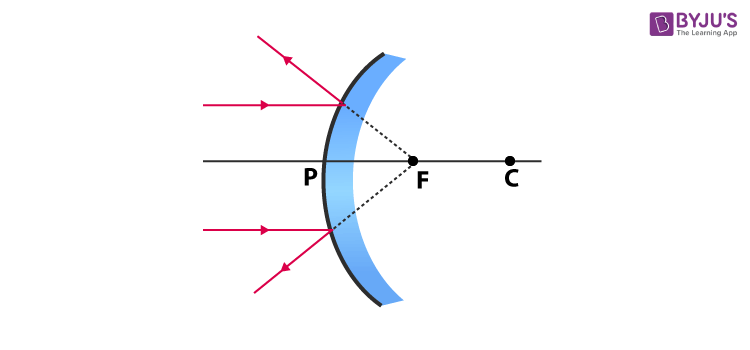b)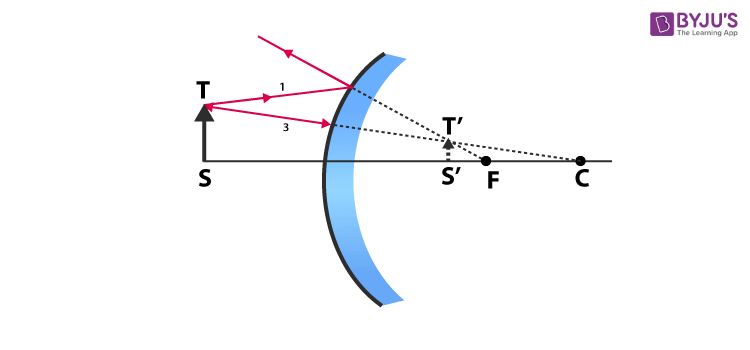c)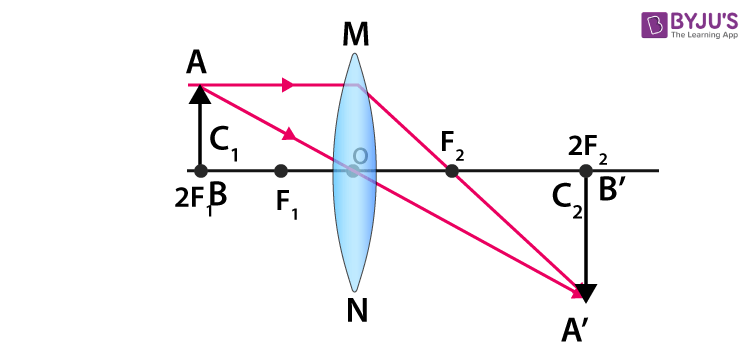d)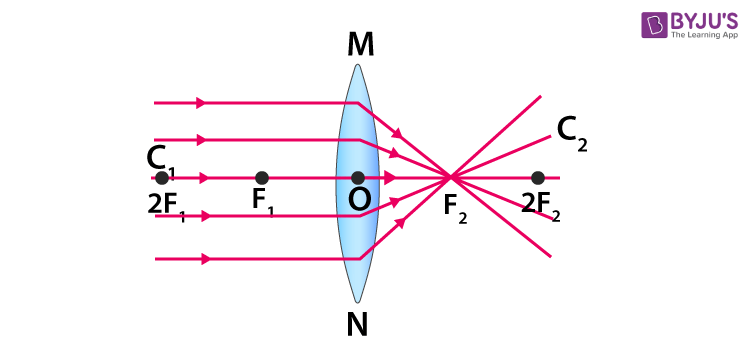e)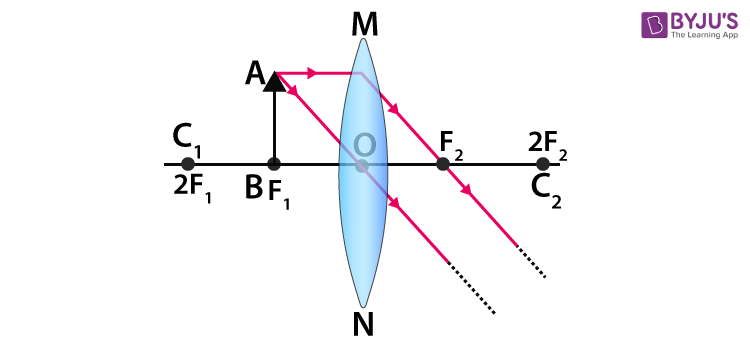32. Write laws of refraction. Explain the same with the help of a ray diagram, when a ray of light passes through a rectangular glass slab.

Soln:

Laws of refraction

1. Incident ray refracted ray and normal at the point of incidence lie in the same plane.
2. The ratio of the sine of incidence and sine of refraction is constant for the given colour and pair of media.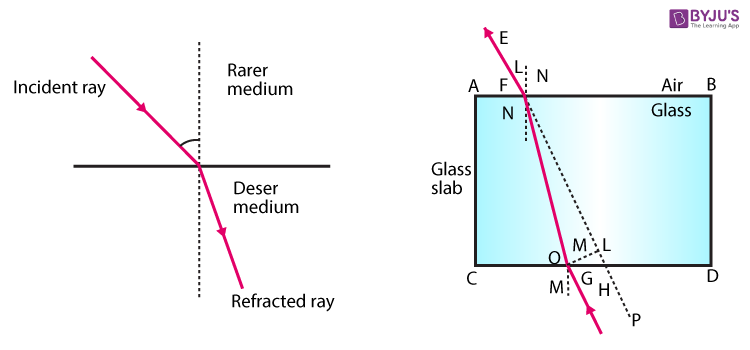• ABCD is a glass slab. EF is an incident ray which is incident on point 0 on the air-glass interface.

• NO is normal and _LEON = : I; which Is angle of incidence.

• NV is normally extended towards the glass slab and ..LV ’00 = Zri; which is the angle of refraction.

• 00′ is refracted ray from surface AB. It behaves like Incident rayon surface CD.

• the ray EF bends when it enters the slab to become 00′.

• MO’ and O’M’ are normal on surface CD.

• GH is the emergent ray.

• ZOO’ Al = Li,; which is the angle of Incidence at surface CD.

• Z.110′ H = Zr,; which is the angle of refraction at surface CD.

• It is observed that EF. NO and 00′ lie in the same plane: which is in accordance with the first law of refraction.

• It is also observed that EF II GH; which means emergent ray is parallel to the incident ray. This happens because the degree of bend at opposite surfaces of the glass slab is the same.

33. Draw ray diagrams showing the image formation by a concave lens when an object is placed

(a) at the focus of the lens

(b) between focus and twice the focal length of the lens

(c) beyond twice the focal length of the lens

Soln:

1. The ray diagram when the object is placed at the focus of the concave lens: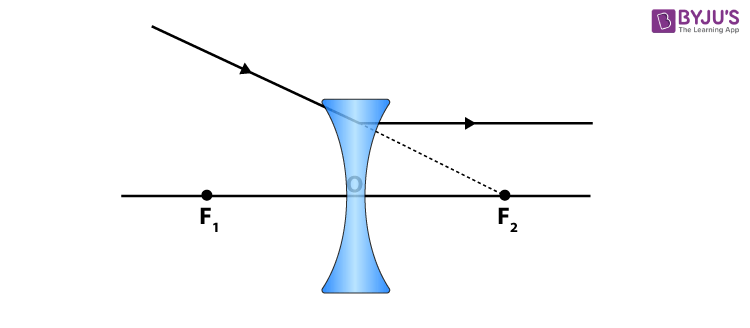1. The ray diagram when the object is placed between focus and twice the length of focal length of the lens: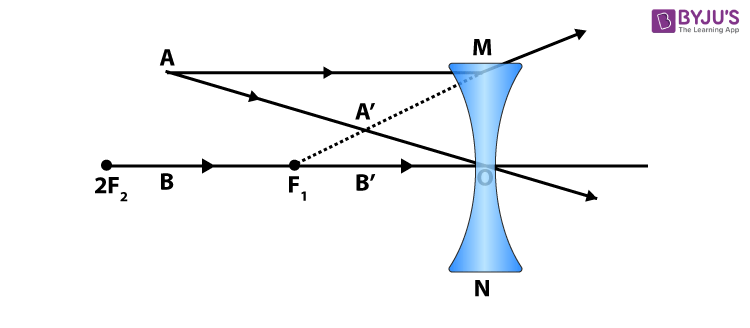(c) Ray diagram when the object is beyond twice the focal length of the concave lens: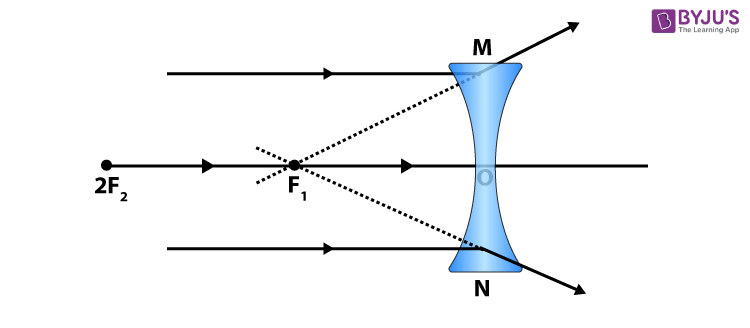34. Draw ray diagrams showing the image formation by a convex mirror when an object is placed

(a) at infinity

(b) at a finite distance from the mirror

Soln:

a) At Infinityb) At infinite distance from the mirror35. The image of a candle flame formed by a lens is obtained on a screen placed on the other side of the lens. If the image is three times the size of the flame and the distance between the lens and image is 80 cm, at what distance should the candle be placed from the lens? What is the nature of the image at a distance of 80 cm and the lens?

Soln:

As the image is obtained on the screen, it is real.

so,

Magnification , m = –3 ,

v = 80 cm

u = ?

As m = v/u

so,

–3 = 80/u,

u = –80/3 cm .

From

1/f = 1/v – 1/u

=1/80 + 3/80

= 4/80

= 1/20

1/f = 1/20cm

so,

f = 20 cm .

The lens is convex and the image formed at 80 cm from the lens is real and inverted.

36. Size of the image of an object by a mirror having a focal length of 20 cm is observed to be reduced to 1/3rd of its size. At what distance the object has been placed from the mirror? What is the nature of the image and the mirror?

Soln:

m = 1/3

Using = 1/v+1/u=1/f

Calculate u;u = – 80 cm.

Image is real and inverted. Mirror is concave.

37. Define power of a lens. What is its unit? One student uses a lens of focal length 50 cm and another of –50 cm. What is the nature of the lens and its power used by each of them?

Soln:

Degree of convergence and divergence provided by a lens is called the power of the lens. Unit of power of lens is Diopter D.

focal length of the lens used by the first student is in positive hence it is a convex lens. The lens of the second student is a concave lens.

p=1/f =1/0.5 =2

Power of lens (first student) =+2

Power of lens (second student) = ).2

38. A student-focussed the image of a candle flame on a white screen using a convex lens. He noted down the position of the candle screen and the lens as under

Position of candle = 12.0 cm

Position of convex lens = 50.0 cm

Position of the screen = 88.0 cm

1. What is the focal length of the convex lens?
2. Where will the image be formed if he shifts the candle towards the lens at a position of 31.0 cm?
3. What will be the nature of the image formed if he further shifts the candle towards the lens?
4. Draw a ray diagram to show the formation of the image in case (iii) as said above.

Soln:

Position of the candle flame = 12.0cm

Position of the lens= 50.0 cm

Position of the screen=88.0 cm

i) u= 50-12= 38 cm.

Image distance v= 88-50= 38cm

Focal length =1/v – 1/u= 1/f

f= 19cm

ii) Object distance u= 50-31= 19 cm

Here

Object distance = focal length

Hence the image is formed at infinity.

iii)

If he further shifts the candle towards the lens. The object comes between F and 0. In this case. Image is virtual, enlarged and erect and is formed on the same side of the lens.

iv)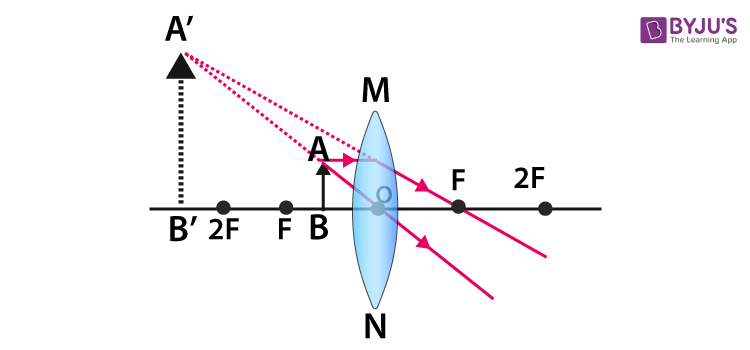Topics covered in NCERT exemplar Class 10 Light Reflection and Refraction:

• Reflection of Light
• Spherical Mirrors
• Image Formation by Spherical Mirrors
• Representation of Images Formed By Spherical Mirrors Using Ray Diagrams Ex
• Sign Convention for Reflection by Spherical Mirrors
• Mirror Formula and Magnification
• Refraction of Light
• Refraction through a Rectangular Glass Slab
• The Refractive Index
• Refraction by Spherical Lenses
• Image Formation by Lenses
• Image Formation in Lenses Using Ray Diagrams
• Sign Convention for Spherical Lenses
• Lens Formula and Magnification
• Power of a Lens.

BYJU’S is the country best online education provider whose innovative approach of teaching and presenting the concepts changing the way students perceive the knowledge. BYJU’S use effective videos, animations and CBSE study materials that help the students learn the topic clearly. To get the benefits provided by BYJU’S log-on to BYJU’S website or download BYJU’S learning App.

## Frequently Asked Questions on NCERT Exemplar Solutions for Class 10 Science Chapter 10

### Does the NCERT Exemplar Solutions for Class 10 Science Chapter 10 help students to grasp the features of reflection and refraction?

Chapter 10 of NCERT Exemplar Solutions for Class 10 Science is an important part in the syllabus. Students need to focus more on the classroom sessions and try to understand what the teacher is explaining during the class hours. The chapters must be studied unit wise and the students must clear their doubts instantly using the solutions available on BYJU’S. The new concepts are also explained in an interactive manner to help students grasp them without any difficulty.

### What are the topics in Chapter 10 of NCERT Exemplar Solutions for Class 10 Science?

Topics covered in NCERT Exemplar Class 10 Light Reflection and Refraction are:
Reflection of Light
Spherical Mirrors
Image Formation by Spherical Mirrors
Representation of Images Formed By Spherical Mirrors Using Ray Diagrams Ex
Sign Convention for Reflection by Spherical Mirrors
Mirror Formula and Magnification
Refraction of Light
Refraction through a Rectangular Glass Slab
The Refractive Index
Refraction by Spherical Lenses
Image Formation by Lenses
Image Formation in Lenses Using Ray Diagrams
Sign Convention for Spherical Lenses
Lens Formula and Magnification
Power of a Lens.

### Why should I use the NCERT Exemplar Solutions for Class 10 Science Chapter 10 PDF from BYJU’S?

Science is one of the important subjects for Class 10 students as most of the concepts are continued in higher levels of education. For this purpose, obtaining a strong foundation of the fundamental concepts is important. Students are recommended to answer the textbook questions using the solutions PDF available on BYJU’S to gain a grip on the important concepts. The PDF of solutions can be downloaded and referred to understand the method of answering complex questions.